Software Engineering for Self-Directed Learners
• # UML

## Introduction

#### What

Can explain/identify class diagrams

UML class diagrams describe the structure (but not the behavior) of an OOP solution. These are possibly the most often used diagrams in the industry and an indispensable tool for an OO programmer.

An example class diagram: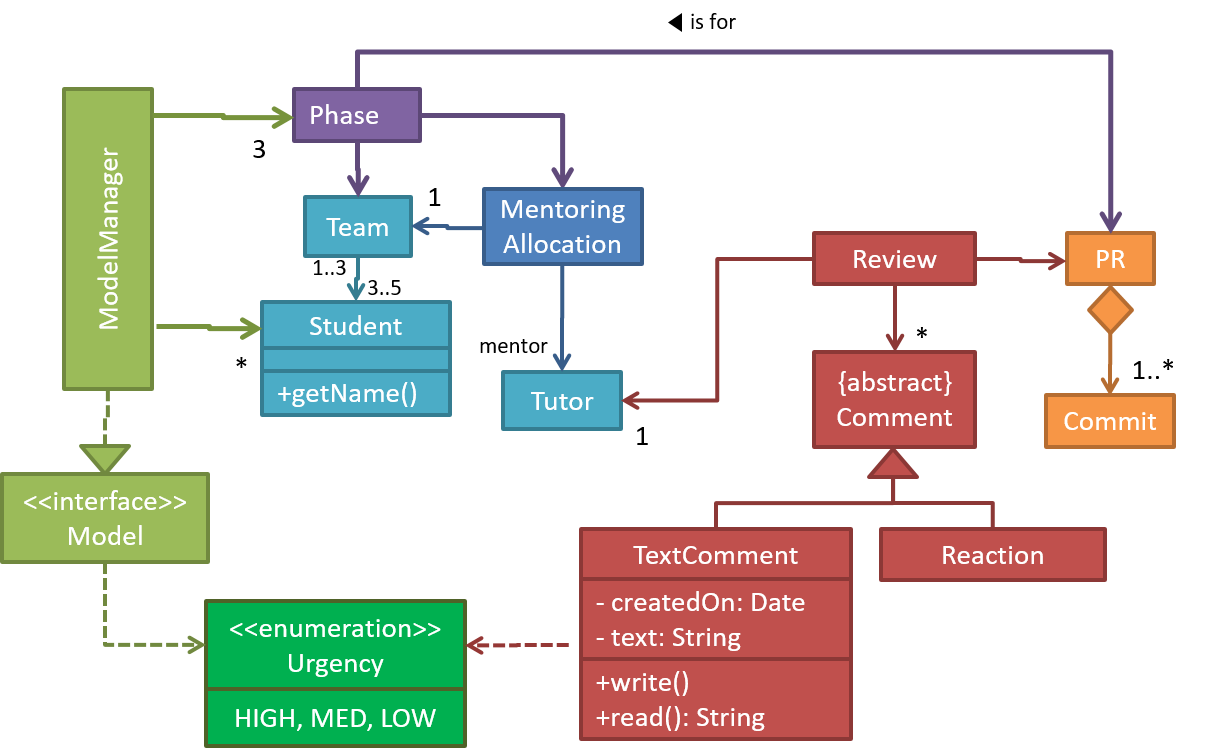## Classes

#### What

Can draw UML classes

The basic UML notations used to represent a class: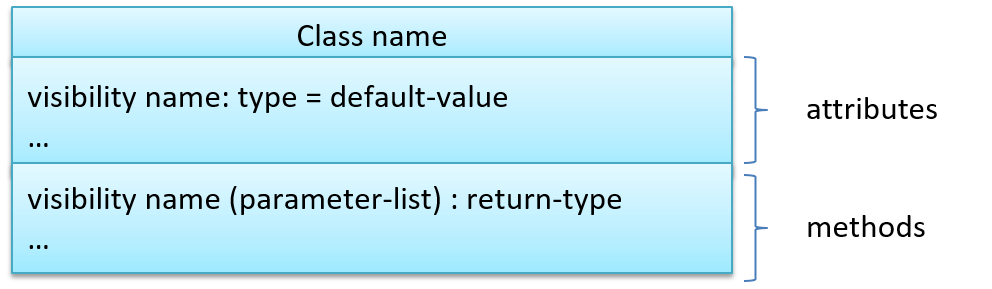A `Table` class shown in UML notation: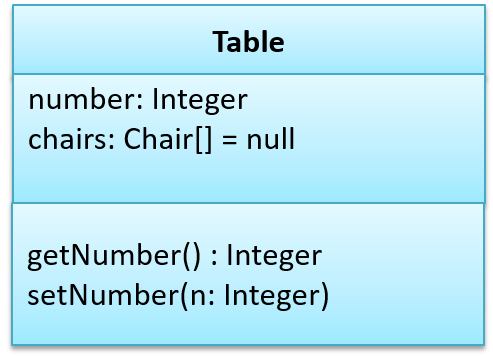Java ``````class Table{ Integer number; Chair[] chairs = null; Integer getNumber(){ //... } void setNumber(Integer n){ //... } } `````` Python ``````class Table: def __init__(self): self.number = 0 self.chairs = [] def get_number(self): # ... def set_number(self, n): # ... ``````

The 'Operations' compartment and/or the 'Attributes' compartment may be omitted if such details are not important for the task at hand. 'Attributes' always appear above the 'Operations' compartment. All operations should be in one compartment rather than each operation in a separate compartment. Same goes for attributes.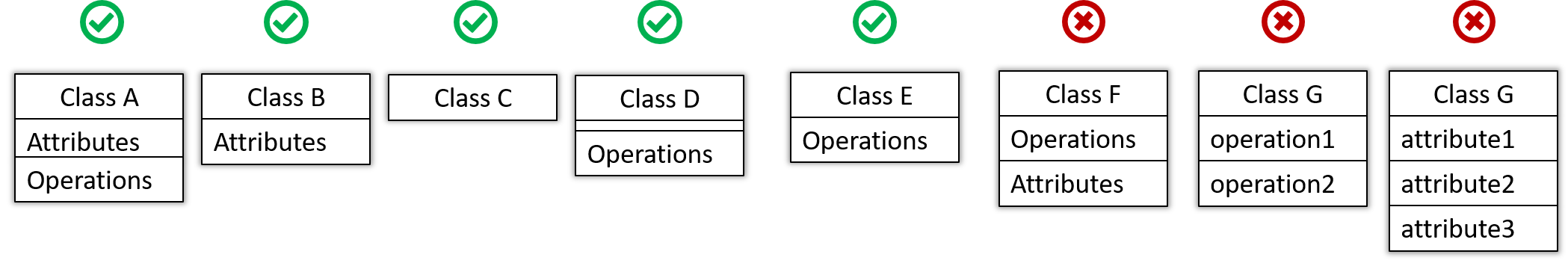The visibility of attributes and operations is used to indicate the level of access allowed for each attribute or operation. The types of visibility and their exact meanings depend on the programming language used. Here are some common visibilities and how they are indicated in a class diagram:

• `+` : public
• `-` : private
• `#` : protected
• `~` : package private
visibility Java Python
`-` private `private` at least two leading underscores (and at most one trailing underscores) in the name
`#` protected `protected` one leading underscore in the name
`+` public `public` all other cases
`~` package private default visibility not applicable

`Table` class with visibilities shown: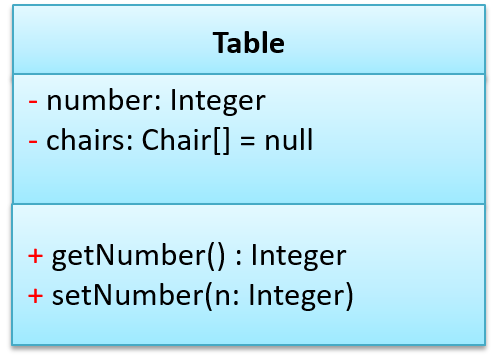Which of these follow the correct UML notation?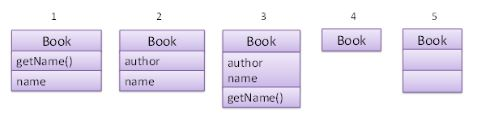• 1
• 2
• 3
• 4
• 5
1. Incorrect : Attributes compartment should be above the Methods compartment.
2. Incorrect : All attributes should be inside the same compartment.
3. Correct
4. Correct : Both Attributes and Methods compartments can be omitted.
5. Correct : The Attributes and Methods compartments can be empty.

Draw a UML diagram to represent the `Car` class as given below. Include visibilities.

``````class Car{

Engine engine;
private List<Wheel> wheels = null;
public String model;

public void drive(int speed){
move(speed);
}

private void move(int speed){
...
}
}
``````

💡 You may omit `self` from method signatures in the class diagram.

``````class Car:

def __init__(self, model):
self._engine = None # type: Engine
self.__wheels = []  # type: a list of Wheel objects
self.model = model  # type: string

def drive(self, speed): # speed is an int
self.move(speed)

def __move(self, speed):
pass
``````

## Associations

#### What

Can interpret simple associations in a class diagram

We use a solid line to show an association between two classes.This example shows an association between the `Admin` class and the `Student` class: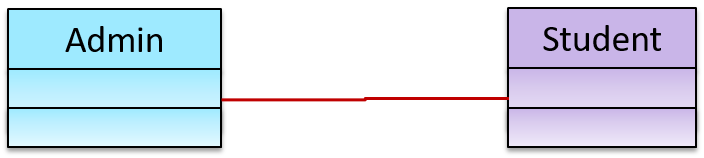Can interpret association navigabilities in class diagrams

We use arrow heads to indication the navigability of an association.

`Logic` is aware of `Minefield`, but `Minefield` is not aware of `Logic`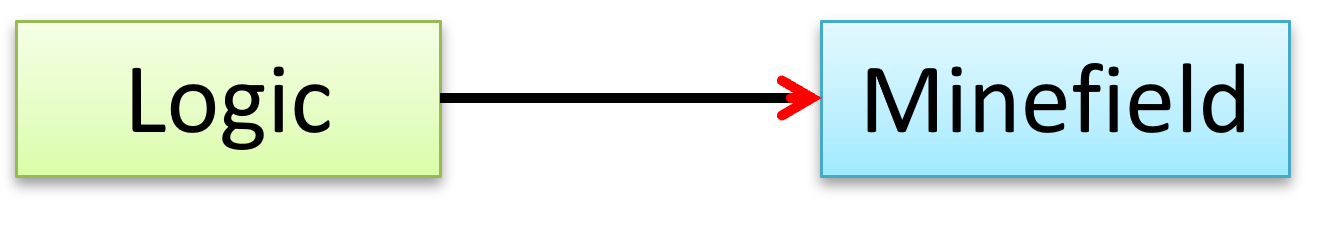``````class Logic{
Minefield minefield;
}

class Minefield{
...
}
``````
``````class Logic:

def __init__(self, minefield):
self.minefield = minefield

# ...

class Minefield:
# ...
``````

Here is an example of a bidirectional navigability; each class is aware of the other.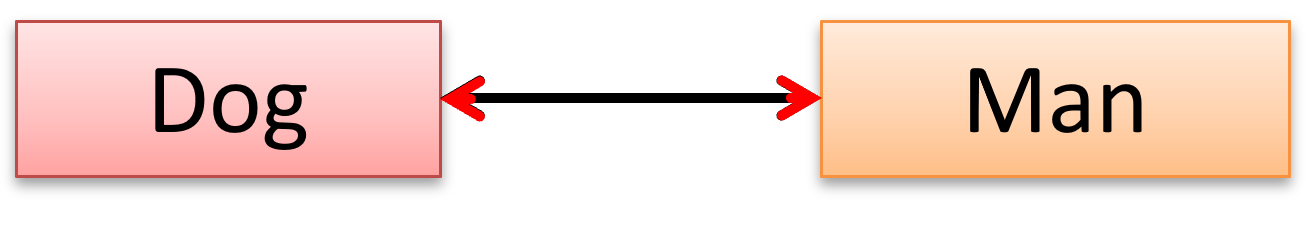Navigability can be shown in class diagrams as well as object diagrams.

According to this object diagram the given `Logic` object is associated with and aware of two `MineField` objects.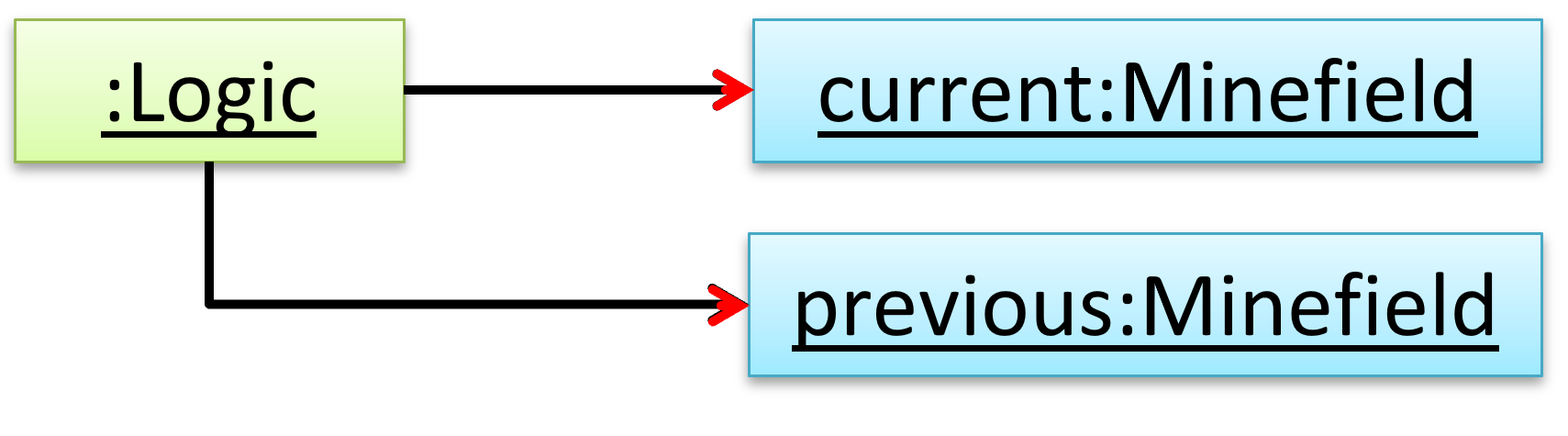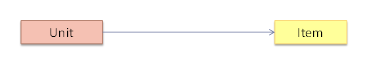(a)

Explanation: The diagram indicates that `Unit` object should know about the `Item` object. In the implementation, the `Unit` object will hold a `Unit` object in one of its variables.

#### Roles

Can explain/use association roles in class diagrams

Association Role labels are used to indicate the role played by the classes in the association.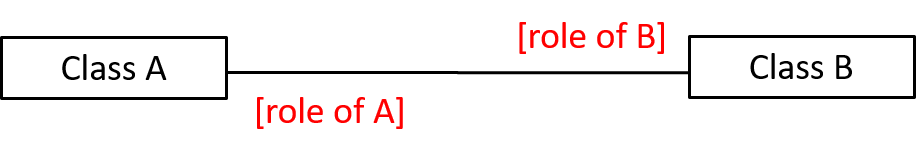This association represents a marriage between a `Man` object and a `Woman` object. The respective roles played by objects of these two classes are `husband` and `wife`.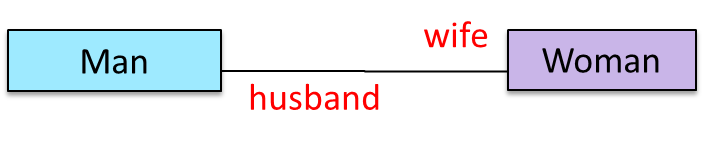Note how the variable names match closely with the association roles.

 Java ``````class Man{ Woman wife; } class Woman{ Man husband; } `````` Python ``````class Man: def __init__(self): self.wife = None # a Woman object class Woman: def __init__(self): self.husband = None # a Man object ``````

The role of `Student` objects in this association is `charges` (i.e. Admin is in charge of students)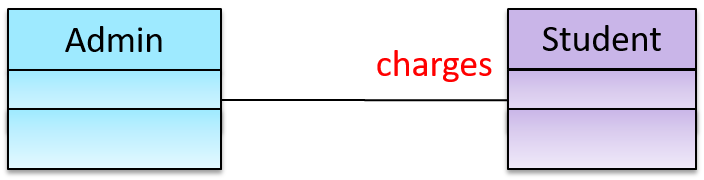Java ``````class Admin{ List charges; } `````` Python ``````class Admin: def __init__(self): self.charges = [] # list of Student objects ``````

#### Labels

Can explain/use association labels in class diagrams

Association labels describe the meaning of the association. The arrow head indicates the direction in which the label is to be read.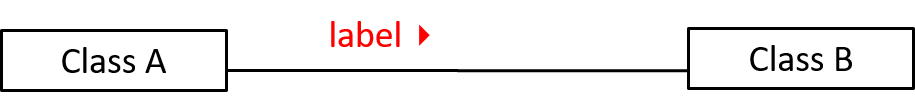In this example, the same association is described using two different labels.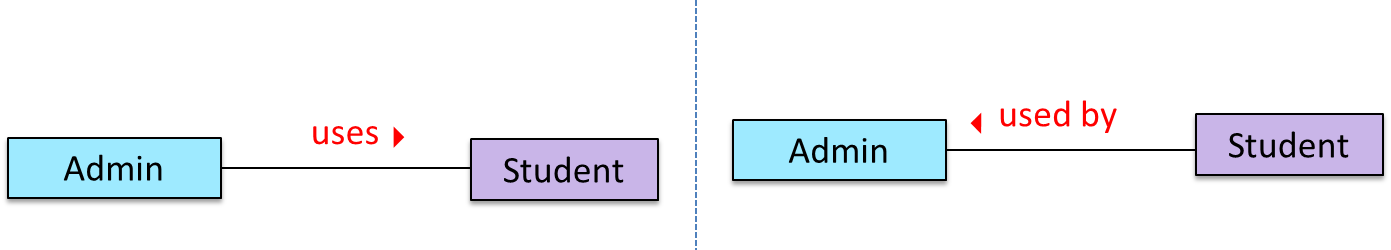• Diagram on the left: `Admin` class is associated with `Student` class because an `Admin` object uses a `Student` object.
• Diagram on the right: `Admin` class is associated with `Student` class because a `Student` object is used by an `Admin` object.

#### Multiplicity

Can explain what is the multiplicity of an association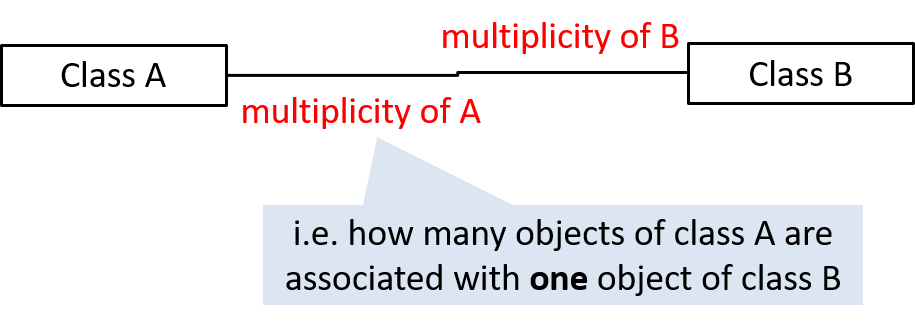Commonly used multiplicities:

• `0..1` : optional, can be linked to 0 or 1 objects
• `1` : compulsory, must be linked to one object at all times.
• `*` : can be linked to 0 or more objects.
• `n..m` : the number of linked objects must be `n` to `m` inclusive

In the diagram below, an `Admin` object administers (in charge of) any number of students but a `Student` object must always be under the charge of exactly one `Admin` objectIn the diagram below,

• Each student must be supervised by exactly one professor. i.e. There cannot be a student who doesn't have a supervisor or has multiple supervisors.
• A professor cannot supervise more than 5 students but can have no students to supervise.
• An admin can handle any number of professors and any number of students, including none.
• A professor/student can be handled by any number of admins, including none.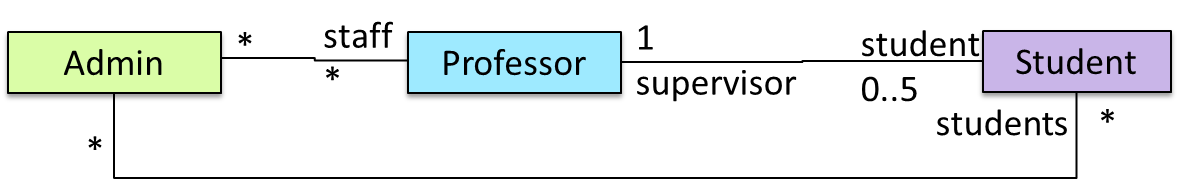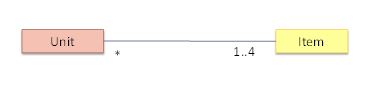• a. A `Unit` object can be linked to any number of `Item` objects
• b. An `Item` object must be linked 1,2,3 or 4 `Unit` objects.
• c. A `Unit` object must be linked 1,2,3, or 4 `Item` objects
• d. A `Item` object can be 0 or more `Unit` objects.

(c)(d)

## Dependencies

#### Dependencies

Can use dependencies in a class diagram

UML uses a dashed arrow to show dependencies.Two examples of dependencies: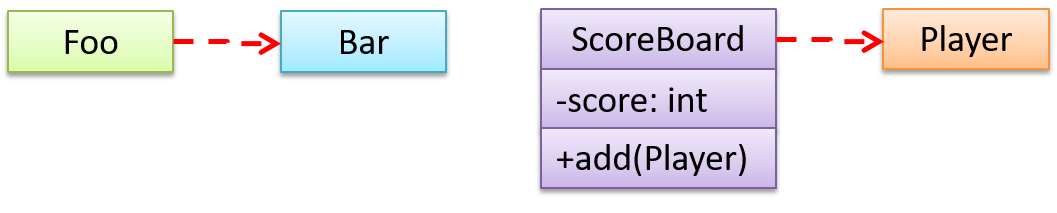## Associations as Attributes

#### Associations as Attributes

Can show an association as an attribute

An association can be shown as an attribute instead of a line.

Association multiplicities and the default value too can be shown as part of the attribute using the following notation. Both are optional.

`name: type [multiplicity] = default value`

The diagram below depicts a multi-player Square Game being played on a board comprising of 100 squares. Each of the squares may be occupied with any number of pieces, each belonging to a certain player.

A `Piece` may or may not be on a `Square`. Note how that association can be replaced by an `isOn` attribute of the `Piece` class. The `isOn` attribute can either be `null` or hold a reference to a `Square` object, matching the `0..1` multiplicity of the association it replaces. The default value is `null`.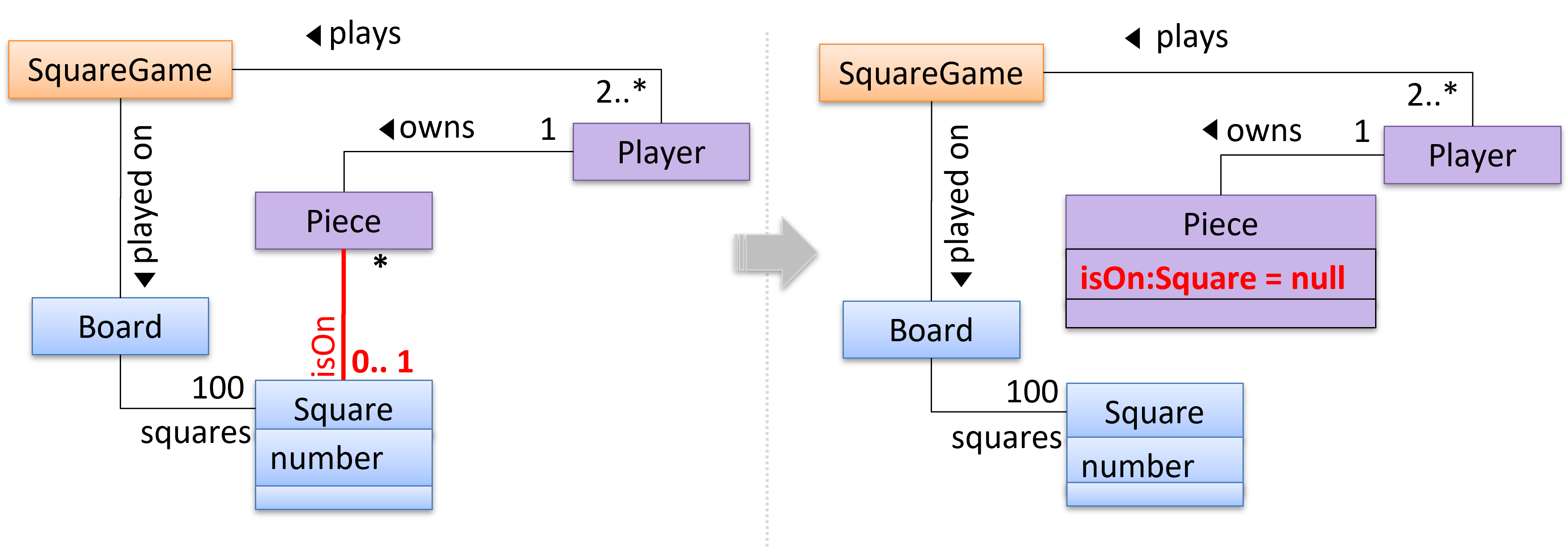The association that a `Board` has 100 `Sqaure`s can be shown in either of these two ways: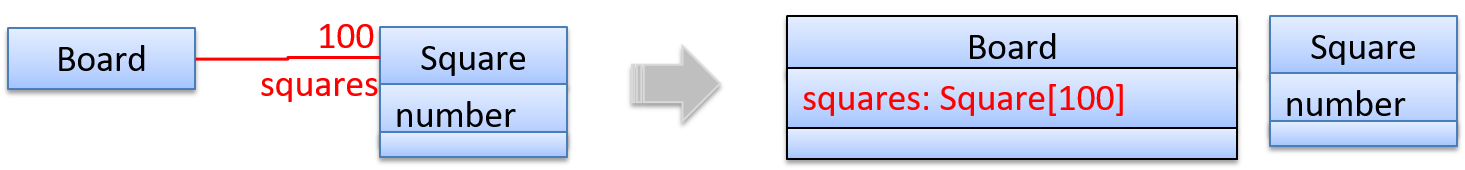## Enumerations

#### What

Can interpret enumerations in class diagrams

Notation: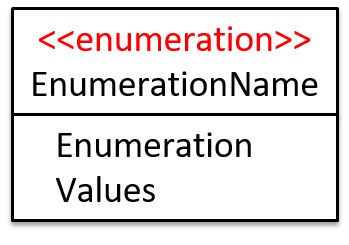In the class diagram below, there are two enumerations in use: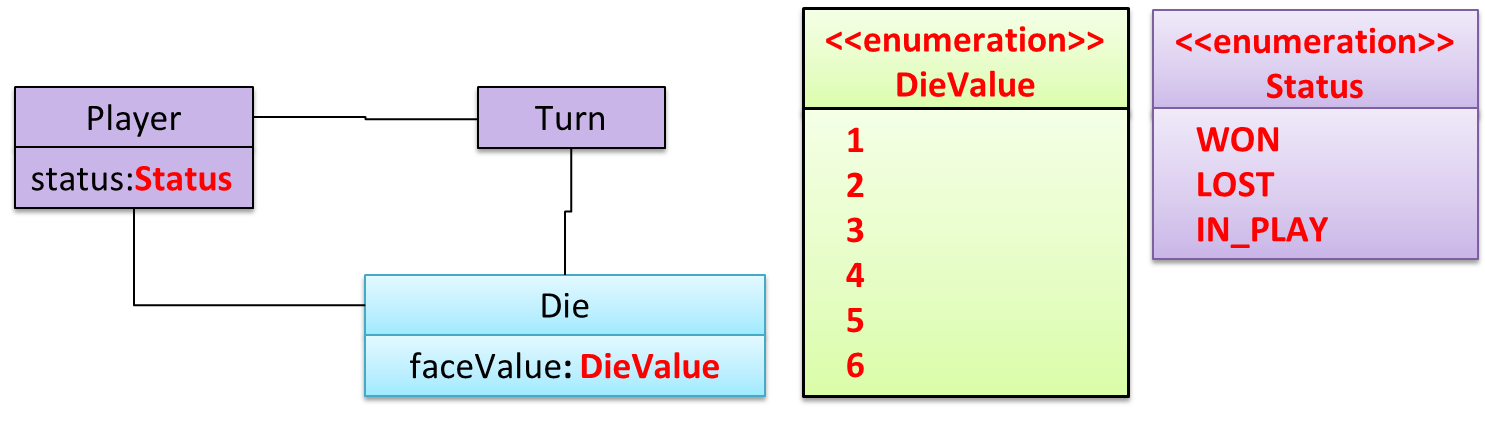Show (in UML notation) an enumeration called `WeekDay` to use when the value can only be `Monday` ... `Friday`.

## Class-Level Members

#### Class-Level Members

Can interpret class-level members in class diagrams

In UML class diagrams, underlines denote class-level attributes and variables.

In the class below, `totalStudents` attribute and the `getTotalStudents` method are class-level.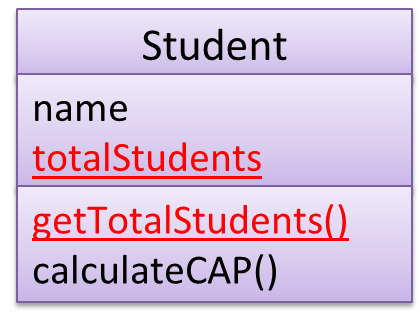## Association Classes

#### Association Classes

Can interpret association classes in class diagrams

Association classes are denoted as a connection to an association link using a dashed line as shown below.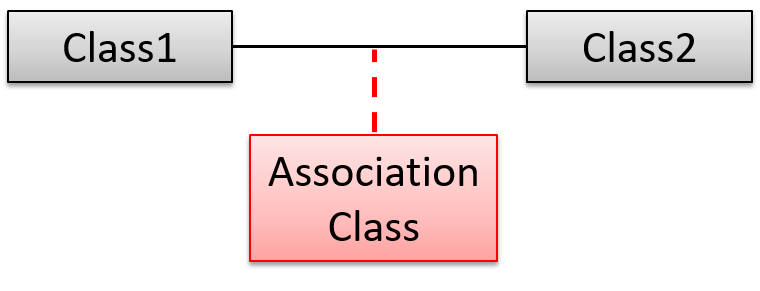In this example `Loan` is an association class because it stores information about the `borrows` association between the `User` and the `Book`.## Composition

#### Composition

Can interpret composition in class diagrams

UML uses a solid diamond symbol to denote composition.

Notation:A `Book` consists of `Chapter` objects. When the `Book` object is destroyed, its `Chapter` objects are destroyed too.## Aggregation

#### Aggregation

Can interpret aggregation in class diagrams

UML uses a hollow diamond is used to indicate an aggregation.

Notation:Example: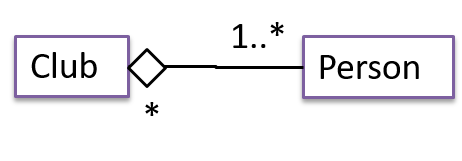Aggregation vs Composition

💡 The distinction between composition (◆) and aggregation (◇) is rather blurred. Martin Fowler’s famous book UML Distilled advocates omitting the aggregation symbol altogether because using it adds more confusion than clarity.

Which one of these is recommended not to use in UML diagrams because it adds more confusion than clarity?

(b)

## Class Inheritance

#### Inheritance

Can interpret class inheritance in class diagrams

You can use a triangle and a solid line (not to be confused with an arrow) to indicate class inheritance.

Notation: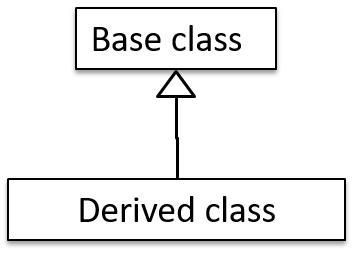Examples: The `Car` class inherits from the `Vehicle` class. The `Cat` and `Dog` classes inherit from the `Pet` class.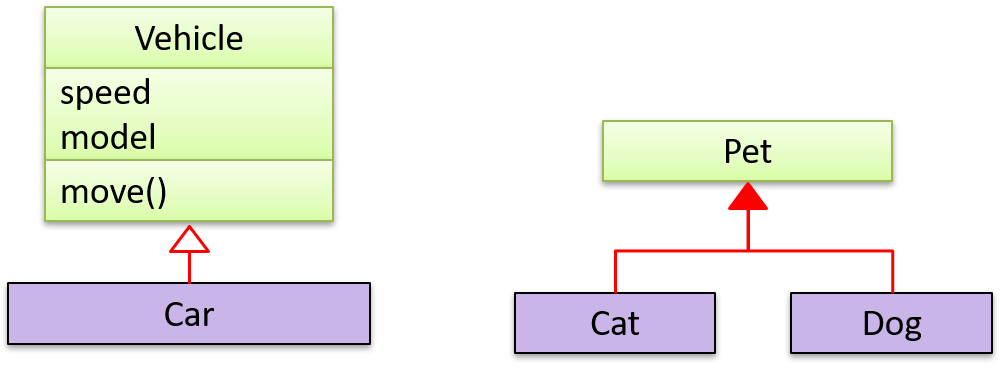## Interfaces

#### Interfaces

Can interpret interfaces in class diagrams

An interface is shown similar to a class with an additional keyword `<< interface >>`. When a class implements an interface, it is shown similar to class inheritance except a dashed line is used instead of a solid line.

The `AcademicStaff` and the `AdminStaff` classes implement the `SalariedStaff` interface.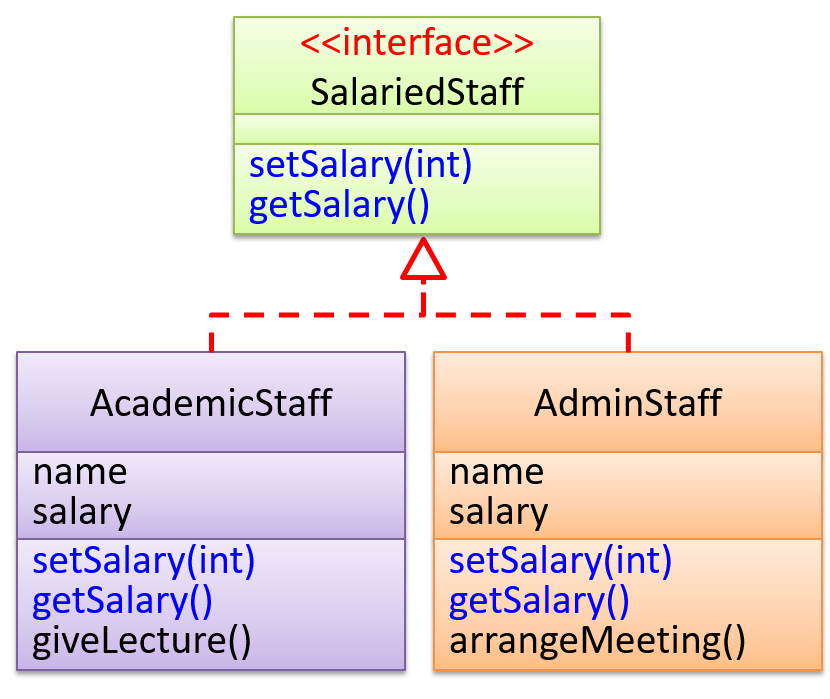## Abstract Classes

#### What

Can interpret abstract classes in class diagrams

You can use italics or `{abstract}` (preferred) keyword to denote abstract classes/methods.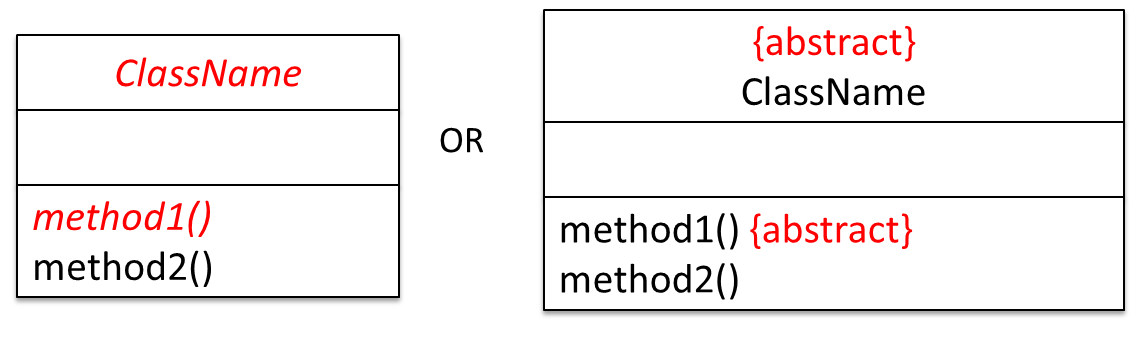Example: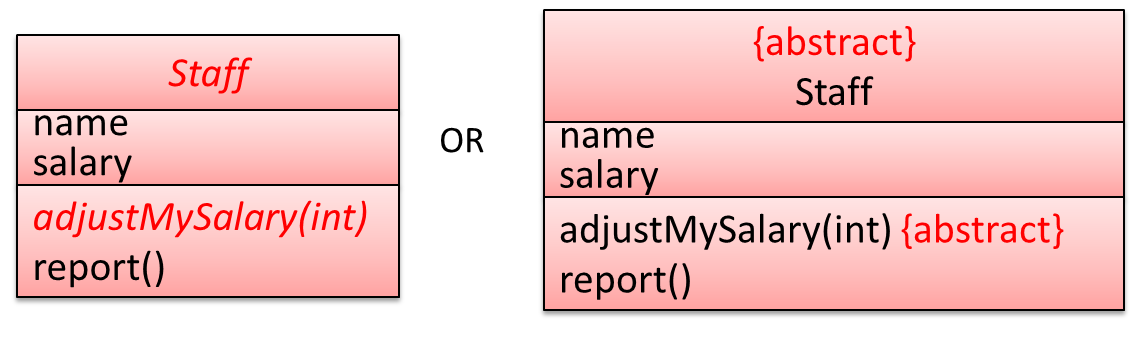## Combine

#### Basic

Can combine different basic aspects of class diagrams

Draw a class diagram for the code below. Also draw an object diagram that will represent the object structure after running `Main#main()`.

• Make the multiplicities as strict as possible without contradicting the code.
• You may omit the `Main` class from both diagrams.
``````
import java.util.ArrayList;
import java.util.List;

public class Main {
public static void main(String[] args) {
Item item1 = new Item();
Item item2 = new Item();
Box box1 = new Box(item1);
Box box2 = new Box(item2);
item2.setMainCard(new Card());
}
}

class Box{
private Item item;
Box (Item item){
this.item = item;
}
}

class Item{
private List<Box> previousBoxes = new ArrayList<>();
private Card mainCard = null;
private Card subCard = null;

void setMainCard(Card card){
this.mainCard = card;
}

void setSubCard(Card card){
this.subCard = card;
}

}
}

class Card{

}
``````

Draw a class diagram for the code below. Also draw an object diagram that will represent the object structure after running the code. Make the multiplicities as strict as possible without contradicting the code.

``````class Box:

def __init__(self, item):
self.__item = item # type: Item

class Item:

def __init__(self):
self.__previous_boxes = [] # type: list of Box objects
self.__main_card = None    # type: Car
self.__sub_card = None     # type: Card

def set_main_card(self, card):
self.__main_card = card # type: Card

def set_sub_card(self, card):
self.__sub_card = card # type: Card

self.__previous_boxes.append(previous_box)

class Card:
pass

item1 = Item();
item2 = Item();
box1 = Box(item1);
box2 = Box(item2);
item2.set_main_card(Card());
``````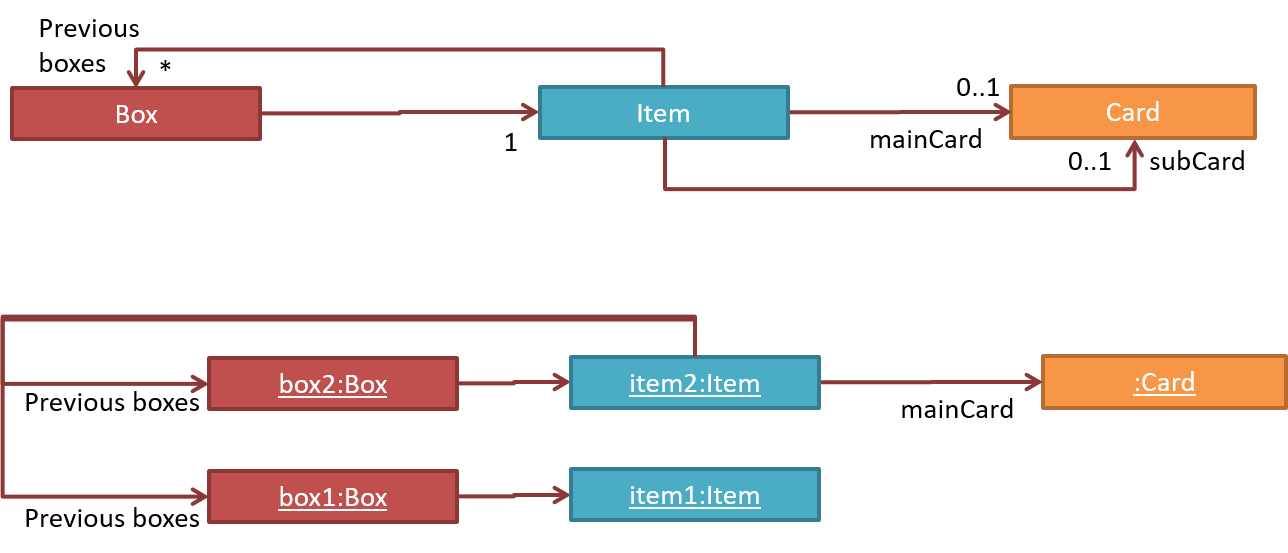Implement the class structure given below: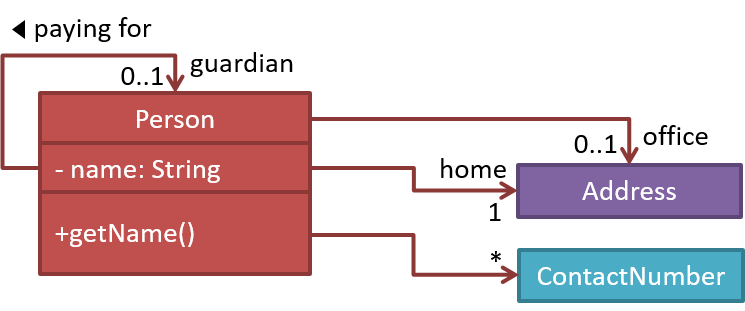Suppose we wrote a program to follow the class structure given in this class diagram:Draw object diagrams to represent the object structures after each of these steps below. Assume that we are trying to minimize the number of total objects.

i.e. apply step 1 → [diagram 1] → apply step 2 on diagram 1 → [diagram 2] and so on.

1. There are no persons.

2. `Alfred` is the Guardian of `Bruce`.

3. `Bruce`'s contact number is the same as `Alfred`'s.

4. `Alfred` is also the guardian of another person. That person lists `Alfred`s home address as his home address as well as office address.

5. `Alfred` has a an office address at `Wayne Industries` building which is different from his home address (i.e. `Bat Cave`).

After step 2, the diagram should be like this: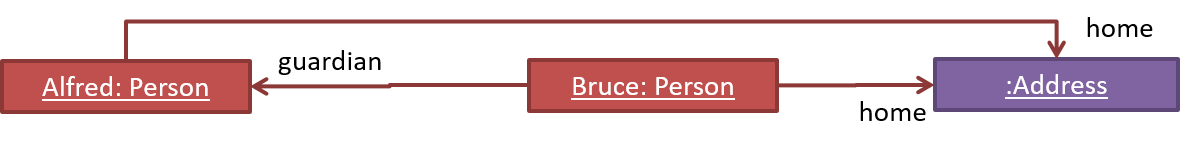## Sequence Diagrams

#### Introduction

Can explain/identify sequence diagrams

A UML sequence diagram captures the interactions between multiple objects for a given scenario.

Consider the code below.

``````class Machine {

Unit producePrototype() {
Unit prototype = new Unit();
for (int i = 0; i < 5; i++) {
prototype.stressTest();
}
return prototype;
}
}

class Unit {

public void stressTest() {

}
}

``````

Here is the sequence diagram to model the interactions for the method call `producePrototype()` on a `Machine` object.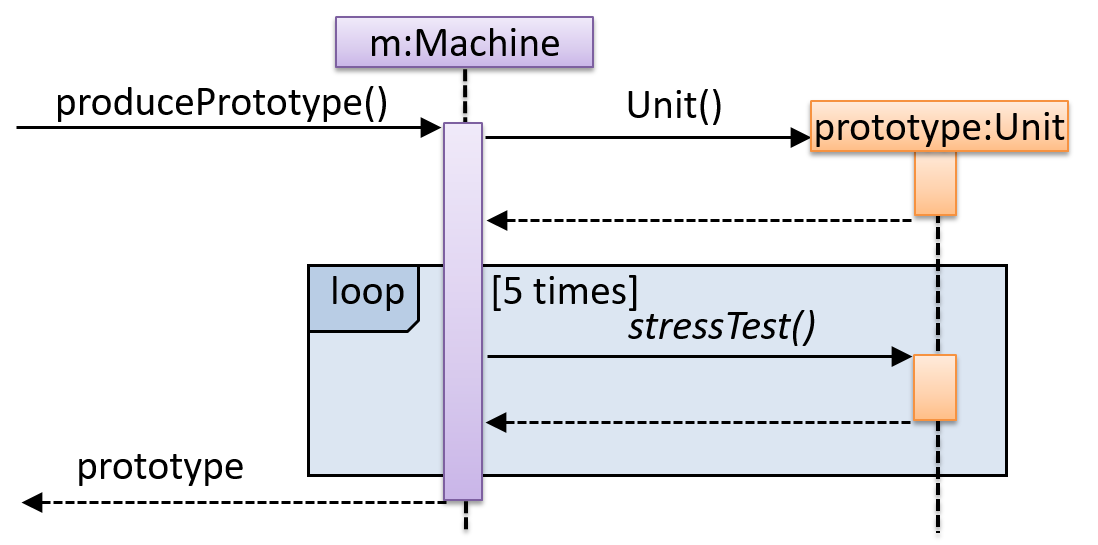#### Basic

Can interpret sequence diagrams with basic notation

Notation: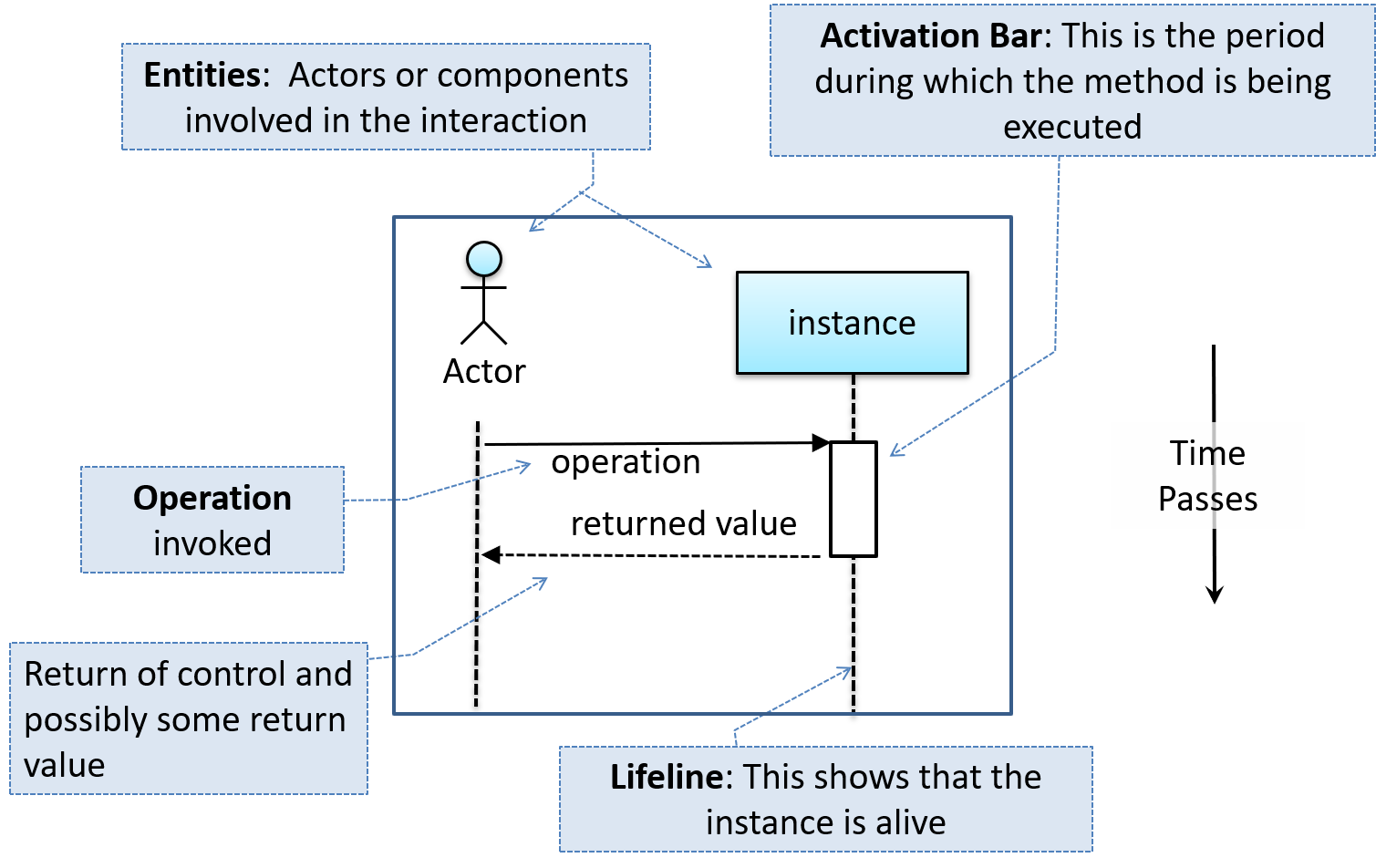This sequence diagram shows some interactions between a human user and the Text UI of a CLI Minesweeper game.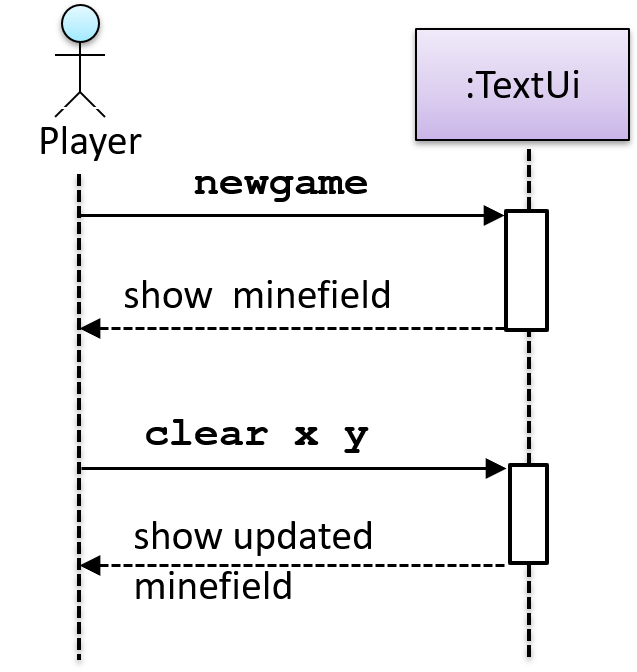The player runs the `newgame` action on the `TextUi` object which results in the `TextUi` showing the minefield to the player. Then, the player runs the `clear x y` command; in response, the `TextUi` object shows the updated minefield.

The `:TextUi` in the above example denotes an unnamed instance of the class TextUi. If there were two instances of `TextUi` in the diagram, they can be distinguished by naming them e.g. `TextUi1:TextUi` and `TextUi2:TextUi`.

Arrows representing method calls should be solid arrows while those representing method returns should be dashed arrows.

Note that unlike in object diagrams, the class/object name is not underlined in sequence diagrams.

[Common notation error] Activation bar too long: The activation bar of a method cannot start before the method call arrives and a method cannot remain active after the method had returned.  In the two sequence diagrams below, the one on the left commits this error because the activation bar starts before the method `Foo#xyz()` is called and remains active after the method returns.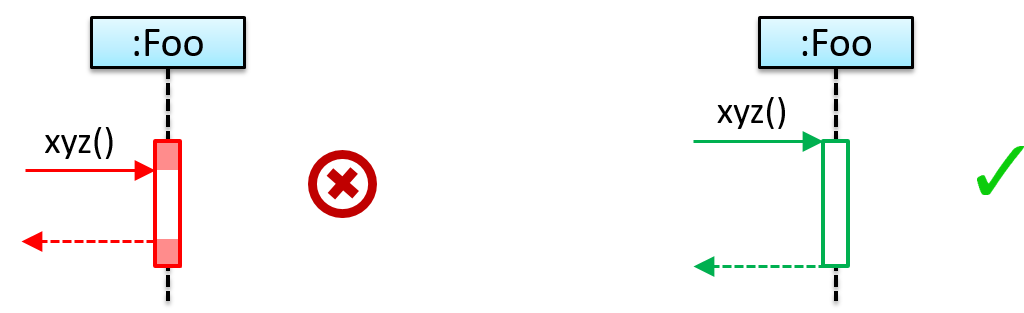[Common notation error] Broken activation bar: The activation bar should remain unbroken from the point the method is called until the method returns.  In the two sequence diagrams below, the one on the left commits this error because the activation bar for the method `Foo#abc()` is not contiguous, but appears as two pieces instead.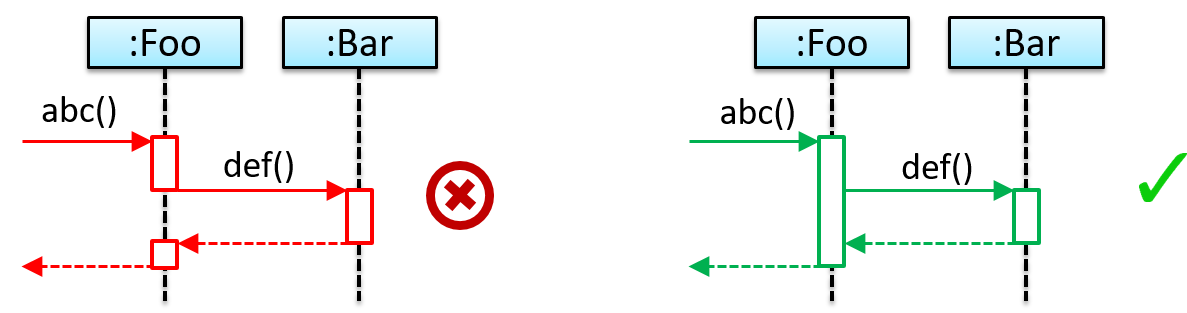#### Object Creation

Can interpret sequence diagrams with object creation

Notation: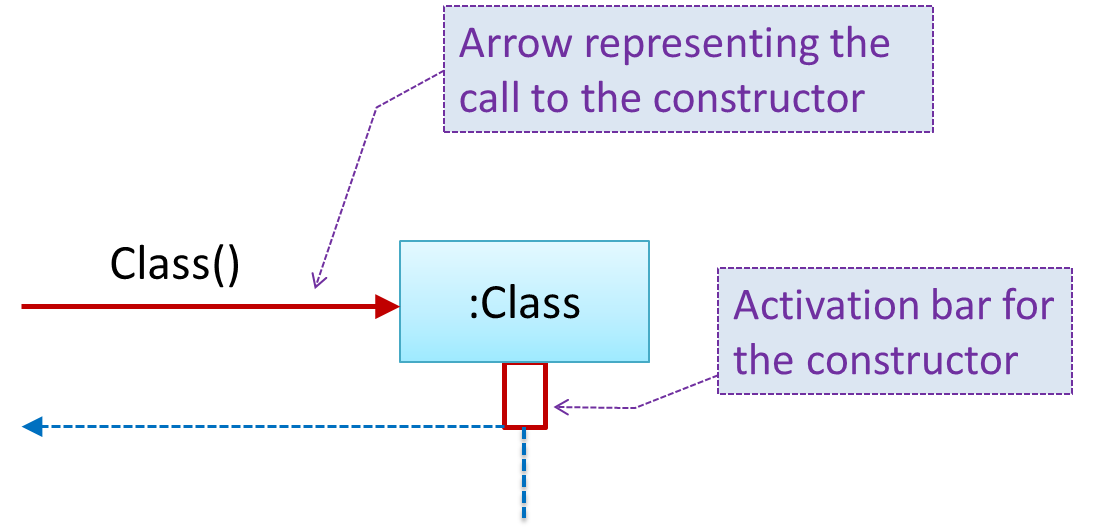• The arrow that represents the constructor arrives at the side of the box representing the instance.
• The activation bar represents the period the constructor is active.

The `Logic` object creates a `Minefield` object.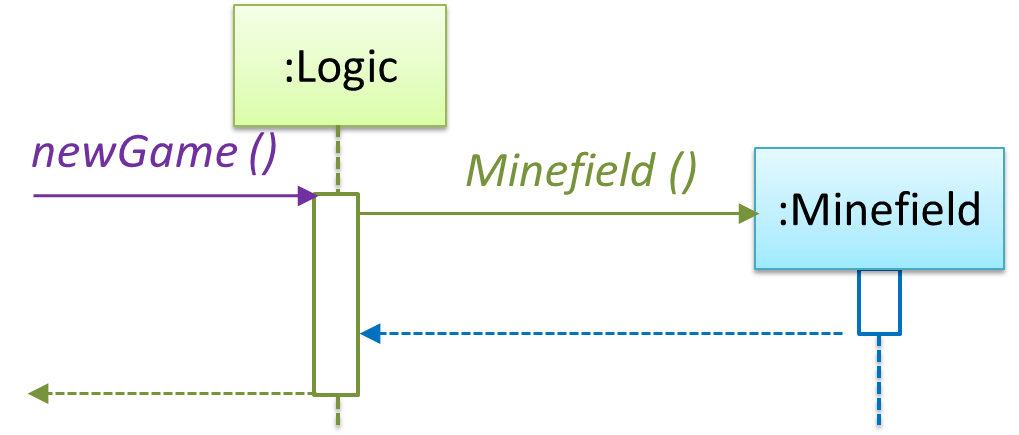#### Object Deletion

Can interpret sequence diagrams with object deletion

UML uses an `X` at the end of the lifeline of an object to show it's deletion.

💡 Although object deletion is not that important in languages such as Java that support automatic memory management, you can still show object deletion in UML diagrams to indicate the point at which the object ceases to be used.

Notation:Note how the diagrams shows the deletion of the `Minefield` object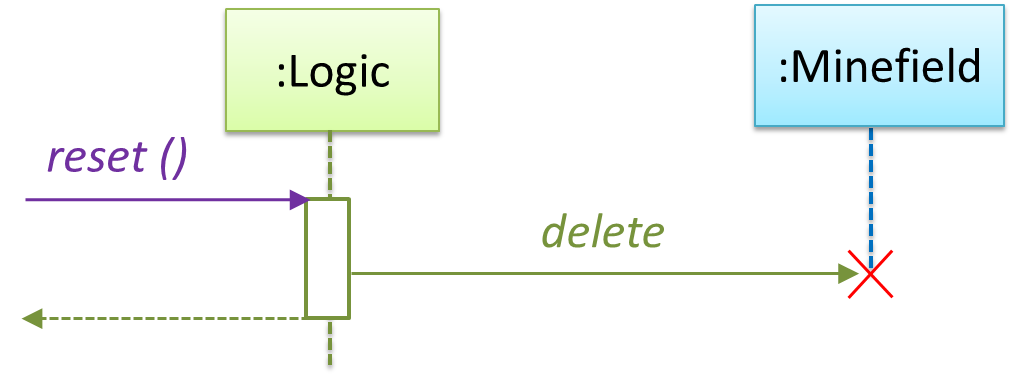#### Loops

Can interpret sequence diagrams with loops

Notation: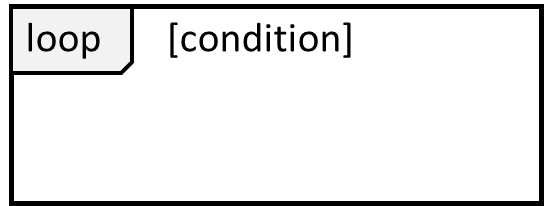The `Player` calls the `mark x,y` command or `clear x y` command repeatedly until the game is won or lost.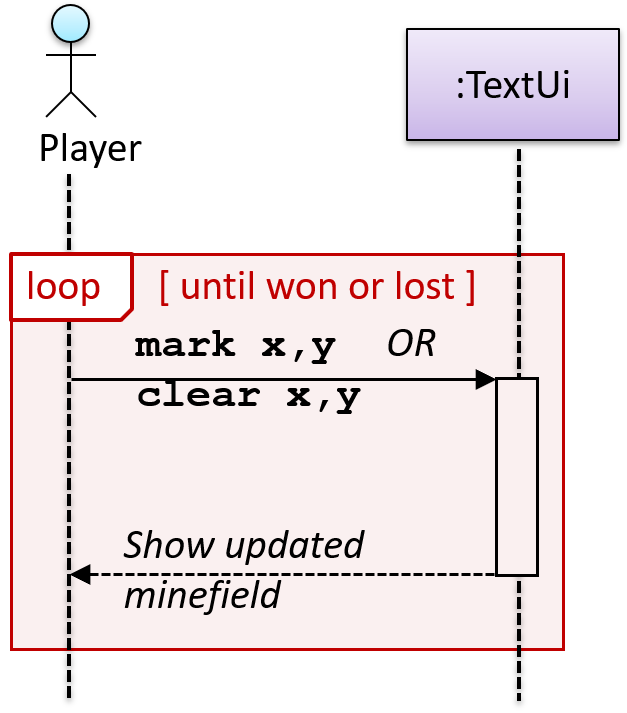#### Self Invocation

Can interpret sequence diagrams with self invocation

UML can show a method of an object calling another of its own methods.

Notation: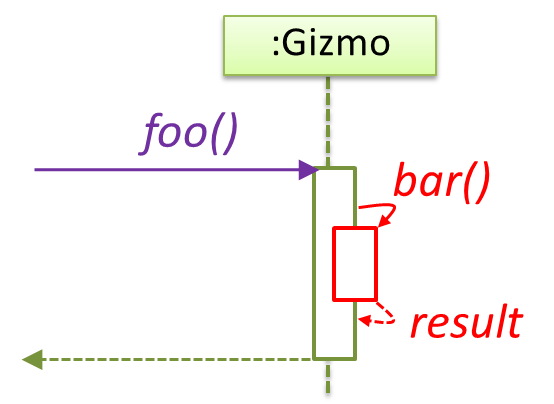The `markCellAt(...)` method of a `Logic` object is calling its own `updateState(...)` method.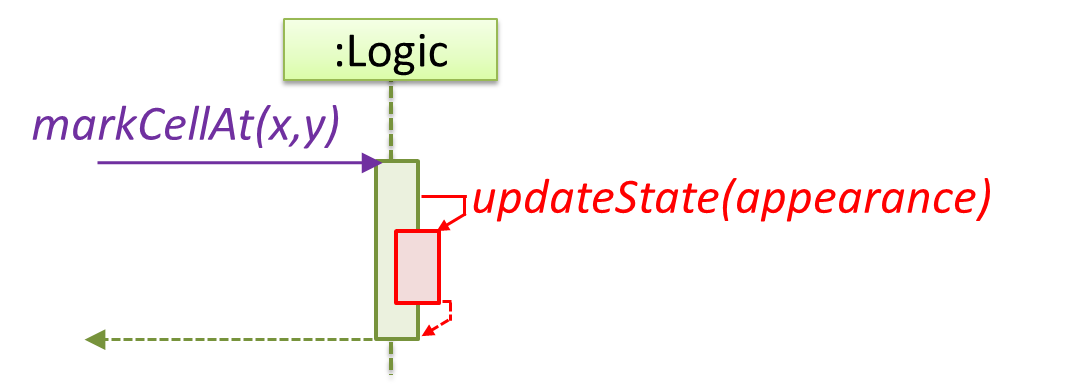In this variation, the `Book#write()` method is calling the `Chapter#getText()` method which in turn does a call back by calling the `getAuthor()` method of the calling object.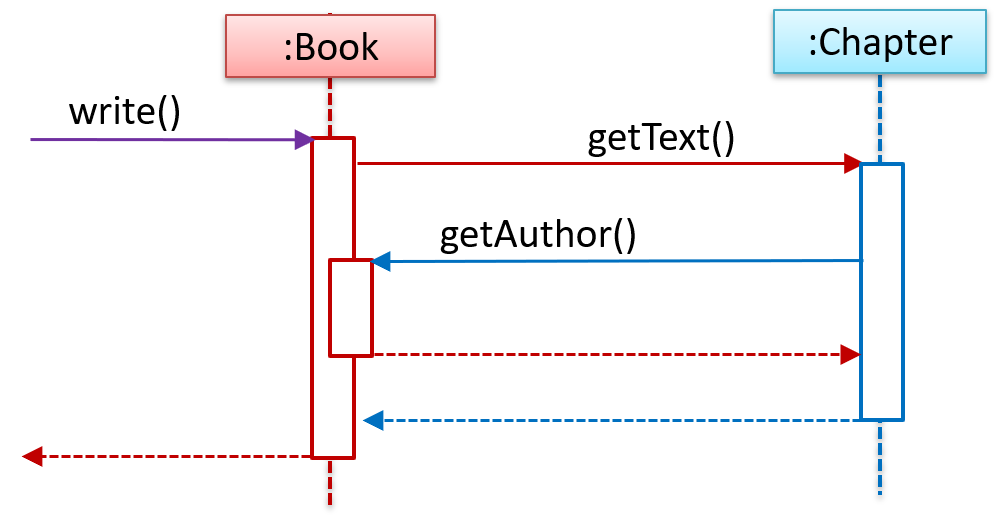#### Alternative Paths

Can interpret sequence diagrams with alternative paths

UML uses `alt` frames to indicate alternative paths.

Notation: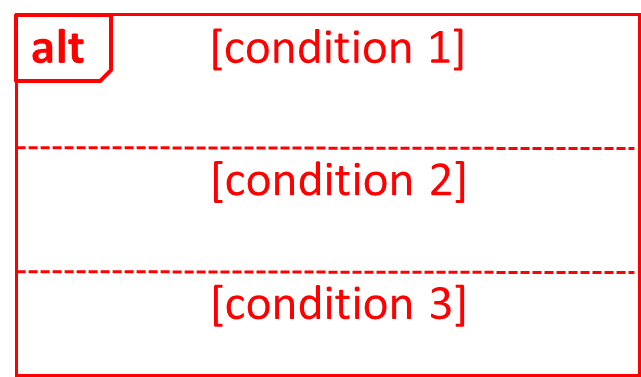`Minefield` calls the `Cell#setMine` if the cell is supposed to be a mined cell, and calls the `Cell:setMineCount(...)` method otherwise.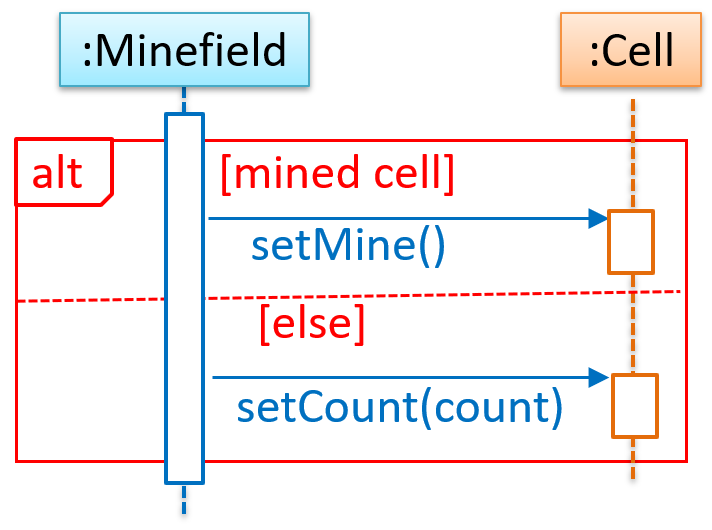#### Optional Paths

Can interpret sequence diagrams with optional paths

UML uses `opt` frames to indicate optional paths.

Notation:`Logic#markCellAt(...)` calls `Timer#start()` only if it is the first move of the player.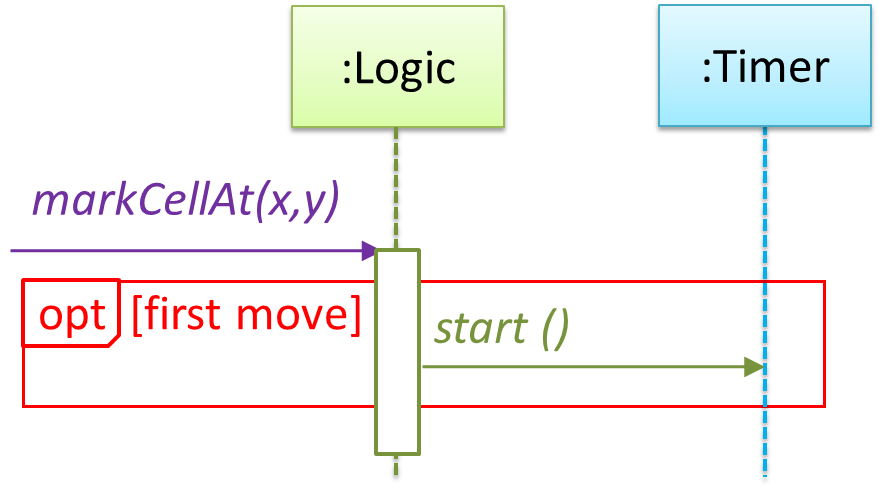#### Parallel Paths

Can interpret sequence diagrams with parallel paths

UML uses `par` frames to indicate parallel paths.

Notation: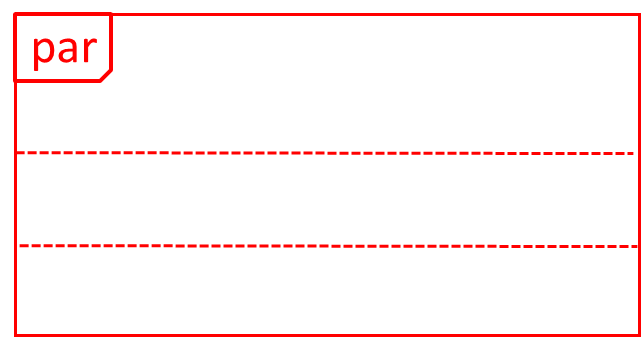`Logic` is calling methods `CloudServer#poll()` and `LocalServer#poll()` in parallel.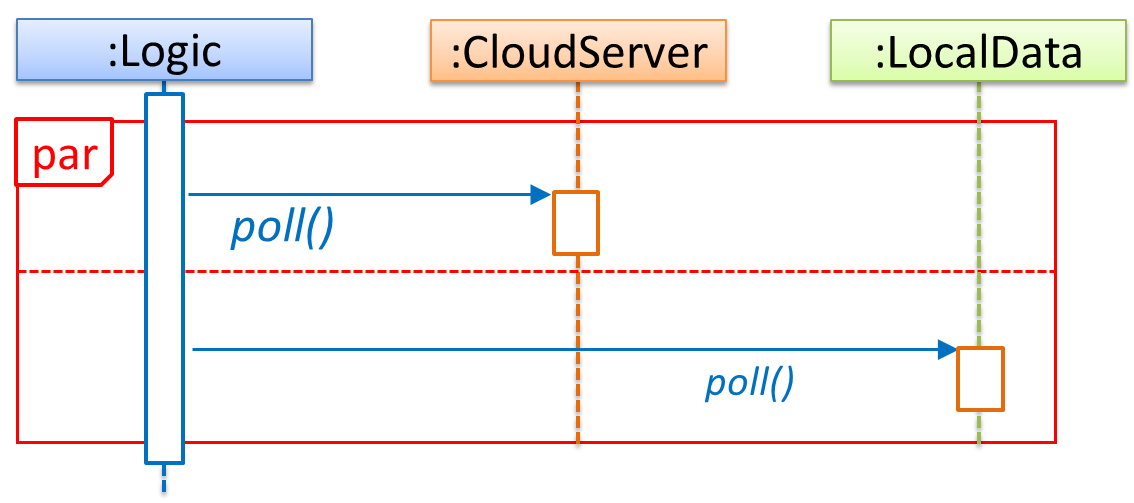💡 If you show parallel paths in a sequence diagram, the corresponding Java implementation is likely to be multi-threaded   because a normal Java program cannot do multiple things at the same time.

#### Reference Frames

Can interpret sequence diagrams with reference frames

UML uses ref frame to allow a segment of the interaction to be omitted and shown as a separate sequence diagram. Reference frames help us to break complicated sequence diagrams into multiple parts or simply to omit details we are not interested in showing.

Notation: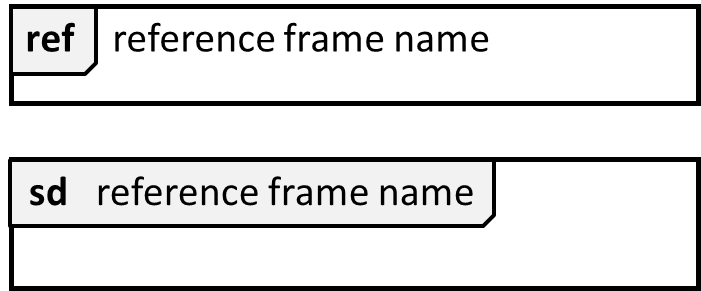The details of the `get minefield appearance` interactions have been omitted from the diagram.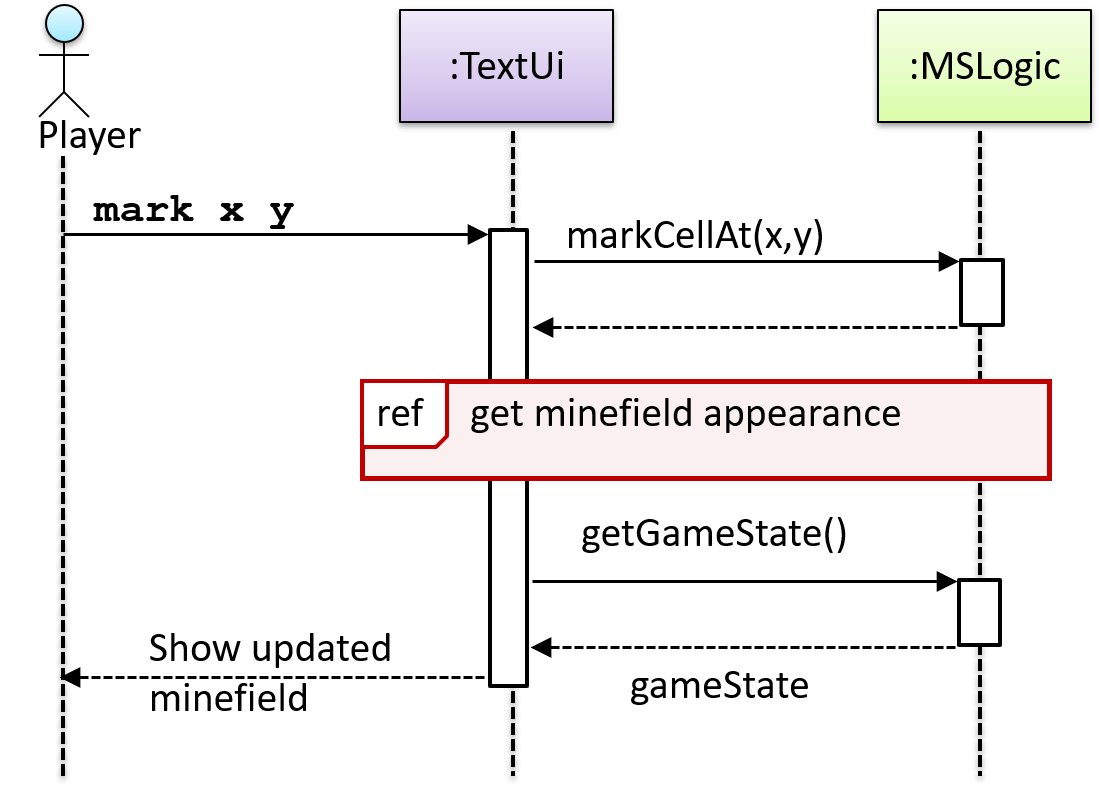Those details are shown in a separate sequence diagram given below.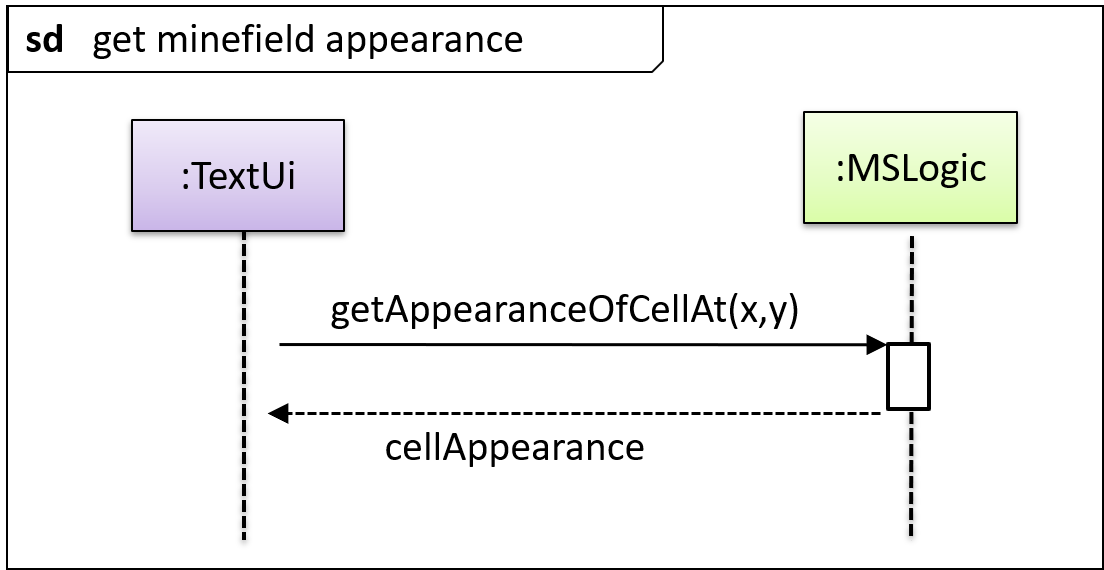#### Minimal Notation

Can interpret sequence diagrams with minimal notation

To reduce clutter, activation bars and return arrows may be omitted if they do not result in ambiguities or loss of information. Informal operation descriptions such as those given in the example below can be used, if more precise details are not required for the task at hand.

A minimal sequence diagram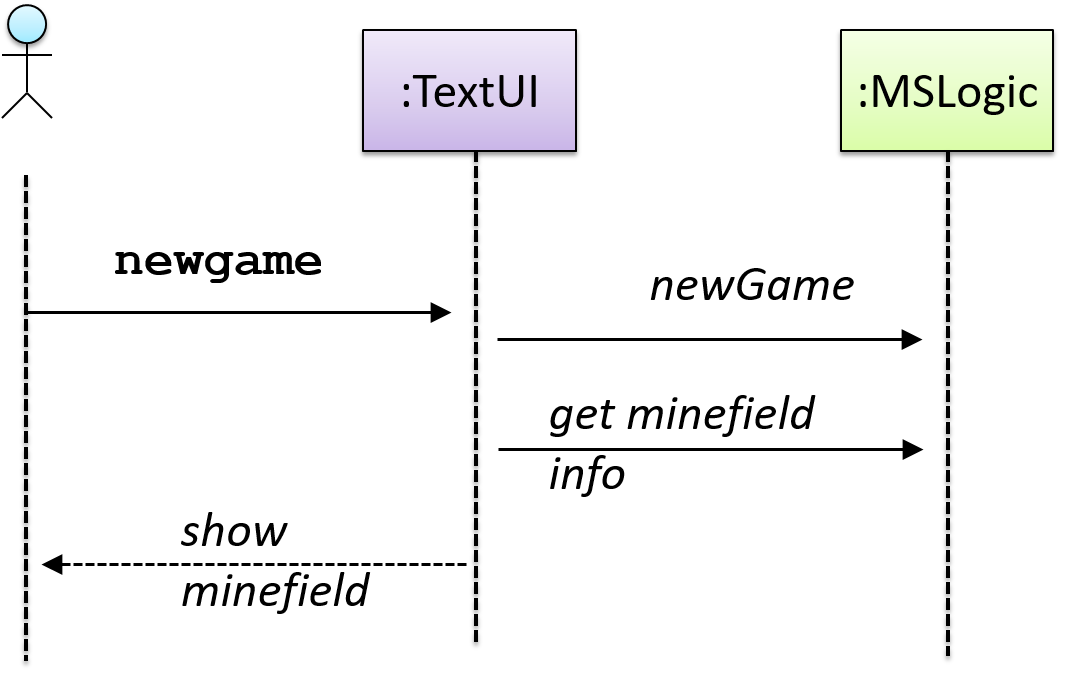## Object Diagrams

#### Introduction

Can explain/identify object diagrams

An object diagram shows an object structure at a given point of time.

An example object diagram: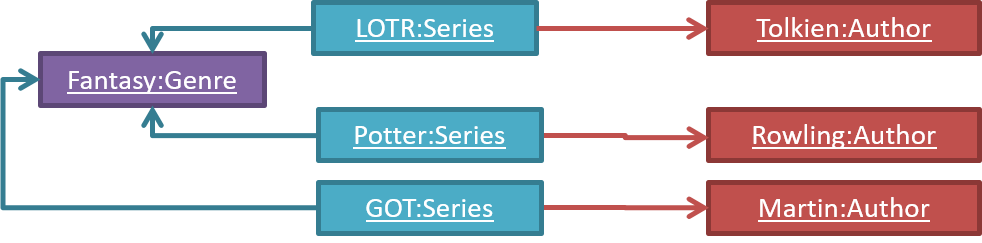#### Objects

Can draw UML objects

Notation: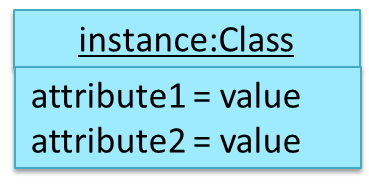Notes:

• The class name and object name e.g. `car1:Car` are underlined.
• `objectName:ClassName` is meant to say 'an instance of `ClassName` identified as `objectName`'.
• Unlike classes, there is no compartment for methods.
• Attributes compartment can be omitted if it is not relevant to the task at hand.
• Object name can be omitted too e.g. `:Car` which is meant to say 'an unnamed instance of a Car object'.

Some example objects: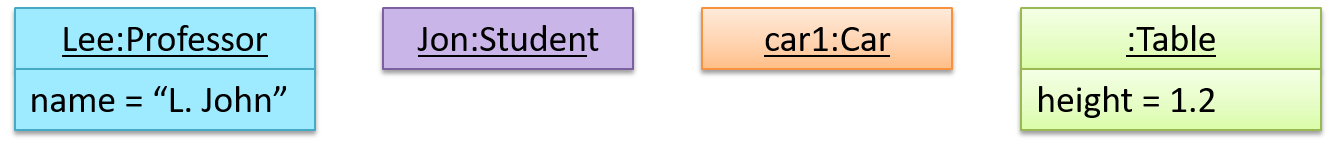Draw a UML diagram to represent the `Car` object created by the following code.

``````class Car{

private double price;
private int speed;

Car(double price, int speed){
//...
}
}

// somewhere else in the code

Car myCar = new Car(13.5, 200);
``````
``````class Car:

def __init__(self, price, speed):
self.__price = price # type: float
self.__speed = speed # type: int

# somewhere else in the code
my_car = Car(13.5, 200);
``````

#### Associations

Can interpret simple associations among objects

A solid line indicates an association between two objects.An example object diagram showing two associations: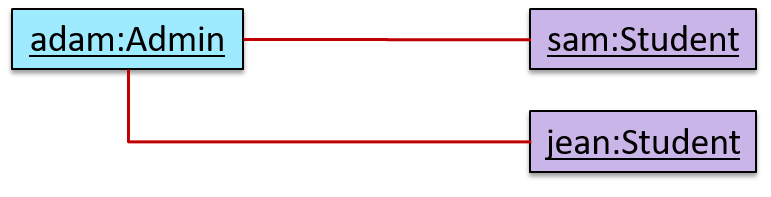## Introduction

#### Introduction

Can explain activity diagrams

UML  activity diagrams (AD) can model workflows.  Flow charts is another type of diagrams that can model workflows. Activity diagrams are the UML equivalent of flow charts.

Unified Modeling Language (UML) is a graphical notation to describe various aspects of a software system. UML is the brainchild of three software modeling specialists James Rumbaugh, Grady Booch and Ivar Jacobson (also known as the Three Amigos). Each of them has developed their own notation for modeling software systems before joining force to create a unified modeling language (hence, the term ‘Unified’ in UML). UML is currently the de facto modeling notation used in the software industry.

An example activity diagram:[source:wikipeida]

## Basic Notations

#### Linear Paths

Can interpret linear paths in activity diagrams

An activity diagram (AD) captures an activity of actions and control flows that makes up the activity.

• An action is a single step in an activity. It is shown as a rectangle with rounded corners.
• A control flow shows the flow of control from one action to the next. It is shown by drawing a line with an arrow-head to show the direction of the flow.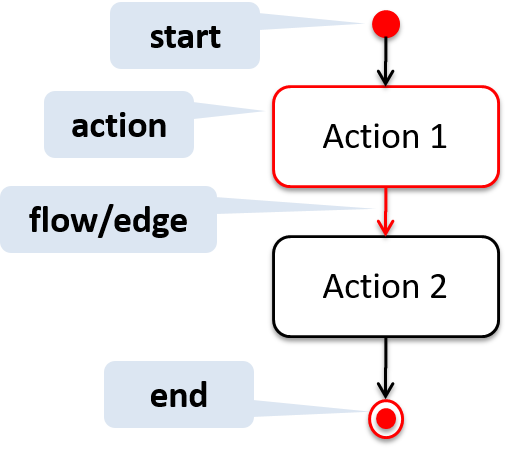Note the slight difference between the start node and the end node which represent the start and the end of the activity, respectively.

This activity diagram shows the action sequence of the activity a passenger rides the bus: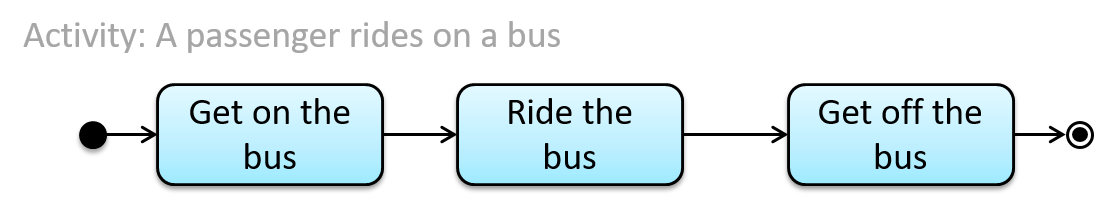Which of these activity diagrams use the correct UML notation?

• i
• ii
• iii
• iv
• v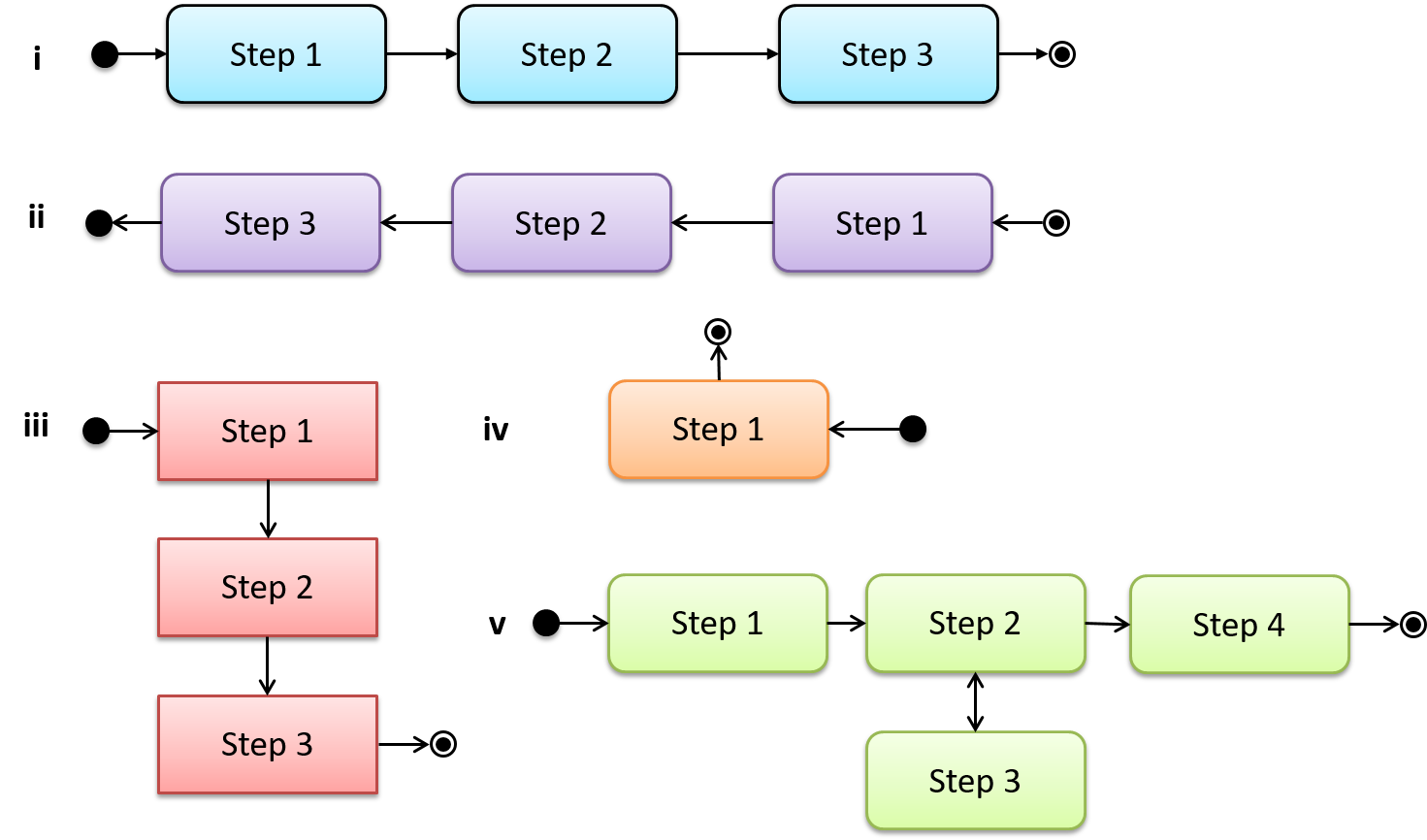• i : 👍 Correct. The arrow-head type does not matter.
• ii : Incorrect. The start and end node notation is swapped.
• iii : Incorrect. Action boxes should have rounded corners.
• iv : 👍 Correct. An activity can have only one action.
• v : Incorrect. There cannot be any double-headed arrows.

#### Alternate Paths

Can interpret alternate paths in activity diagrams

A branch node shows the start of alternate paths. Each control flow exiting a branch node has a guard condition : a boolean condition that should be true for execution to take that path. Only one of the guard condition can be true at any time.

A merge node shows the end of alternate paths.

Both branch nodes and merge nodes are diamond shapes. Guard conditions must be in square brackets.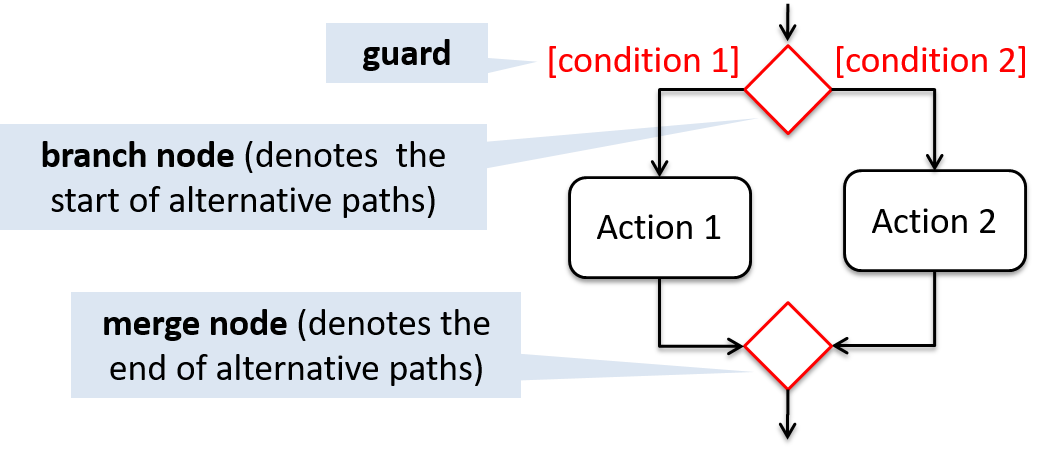The AD below shows alternate paths involved in the workflow of the activity shop for product: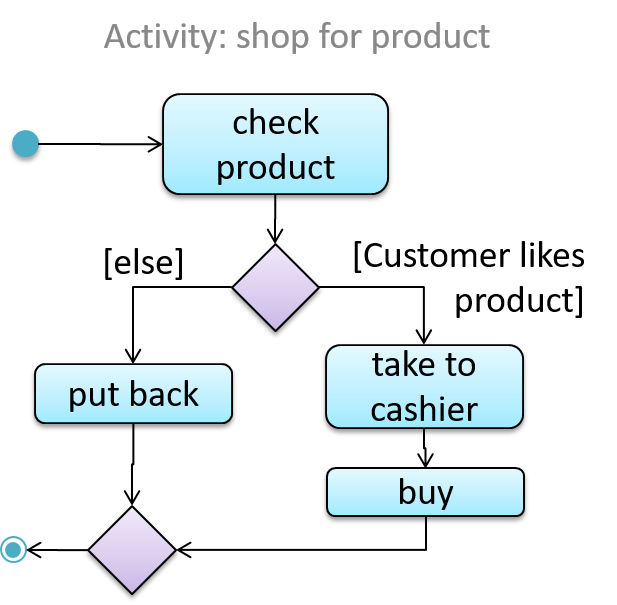Which of these activity diagrams use the correct UML notation?

• i
• ii
• iii
• iv
• v
• vi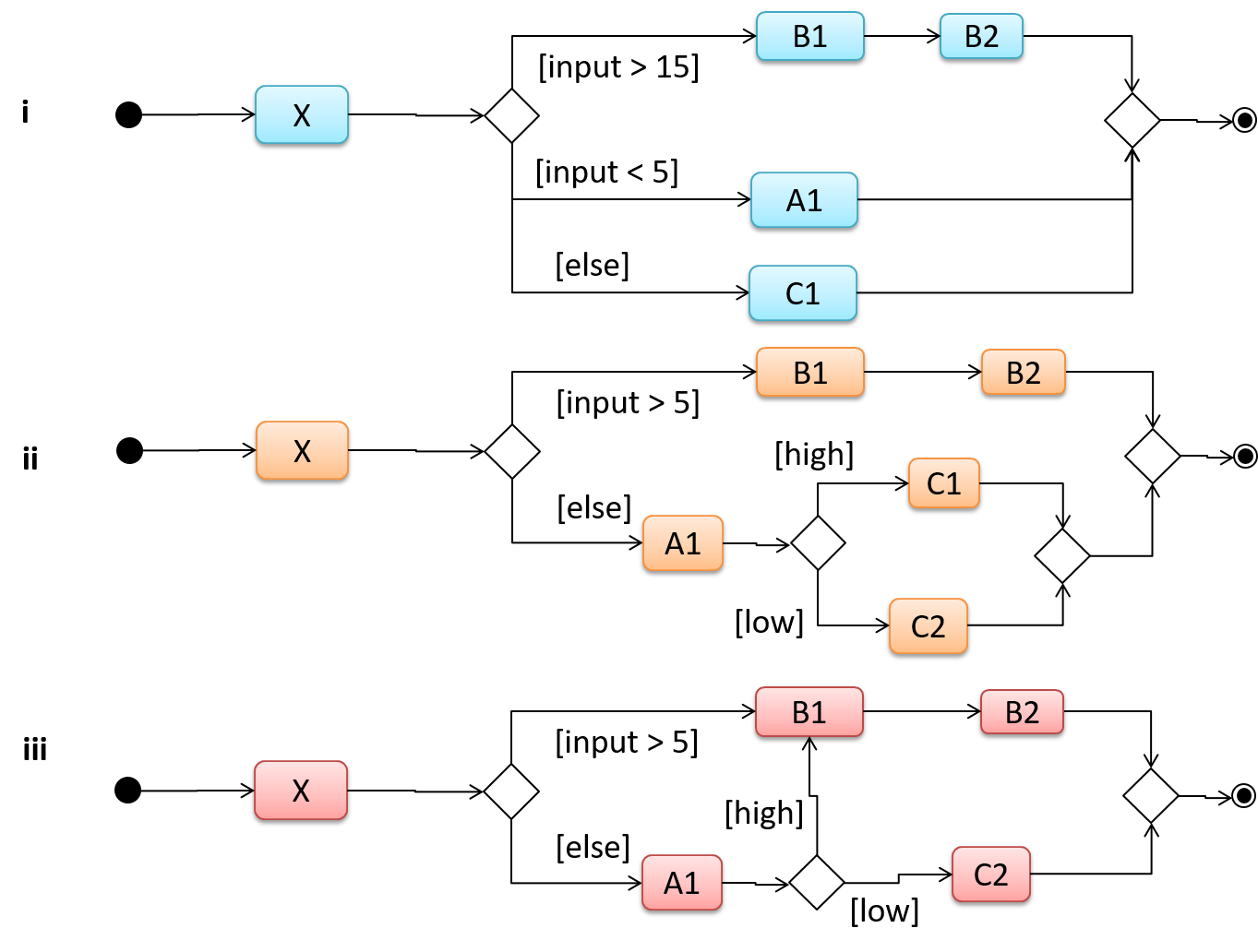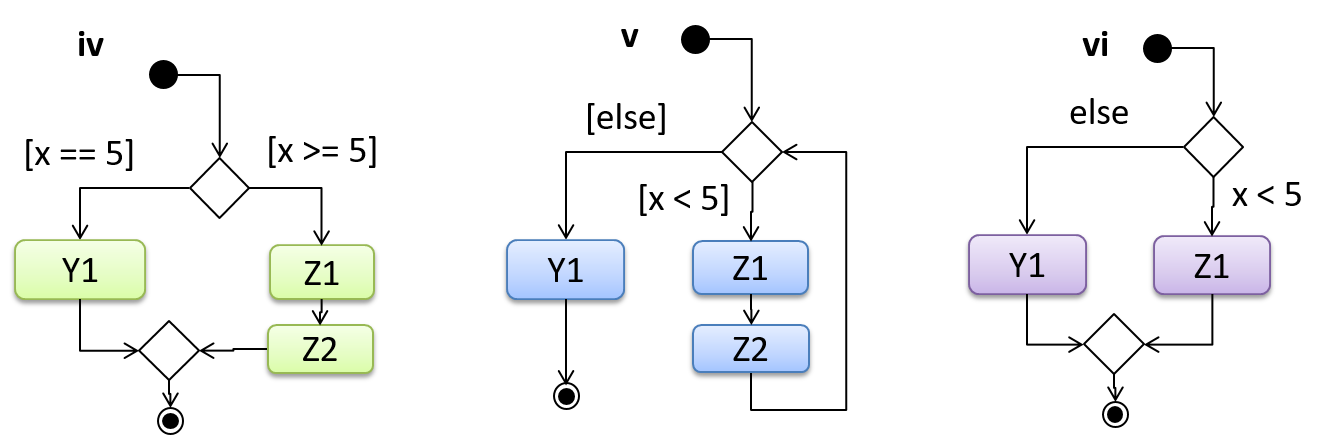• i : 👍 Correct. There can be more than two alternate paths.
• ii : 👍 Correct. An alternate path can divide into more branches.
• iii : 👍 Correct. A branch can join other branches.
• iv : Incorrect. At `x=5` both guard conditions become true.
• v : 👍 Correct. It is fine for a branch to form a loop by going back to the original branch node.
• iv : Incorrect. Guard conditions should be given in square brackets.

#### Parallel Paths

Can interpret parallel paths in activity diagrams

Fork nodes indicate the start of concurrent flows of control.

Join nodes indicate the end of parallel paths.

Both have the same notation: a bar.

In a set of parallel paths, execution along all parallel paths should be complete before the execution can start on the outgoing control flow of the join.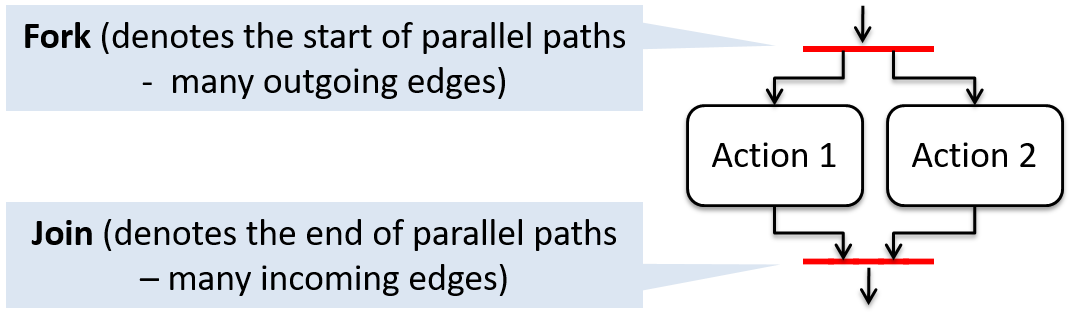In this activity diagram (from an online shop website) the actions User browsers products and System records browsing data happen in parallel. Both of them need to finish before the log out action can take place.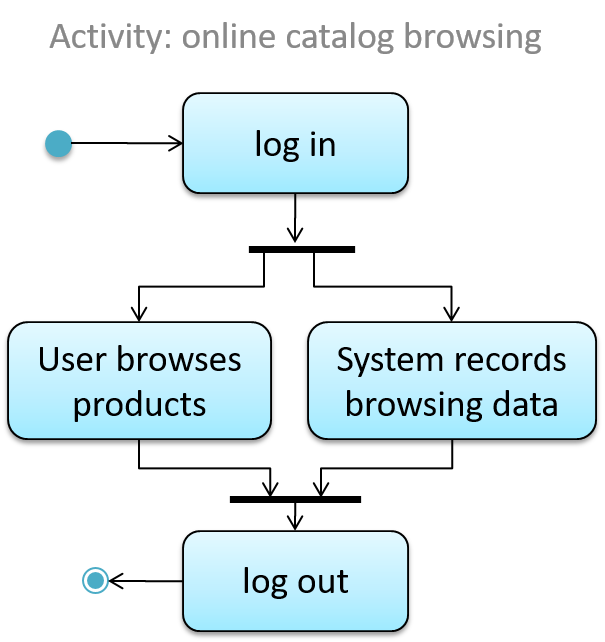Which of these activity diagrams use the correct UML notation?

• i
• ii
• iii
• iv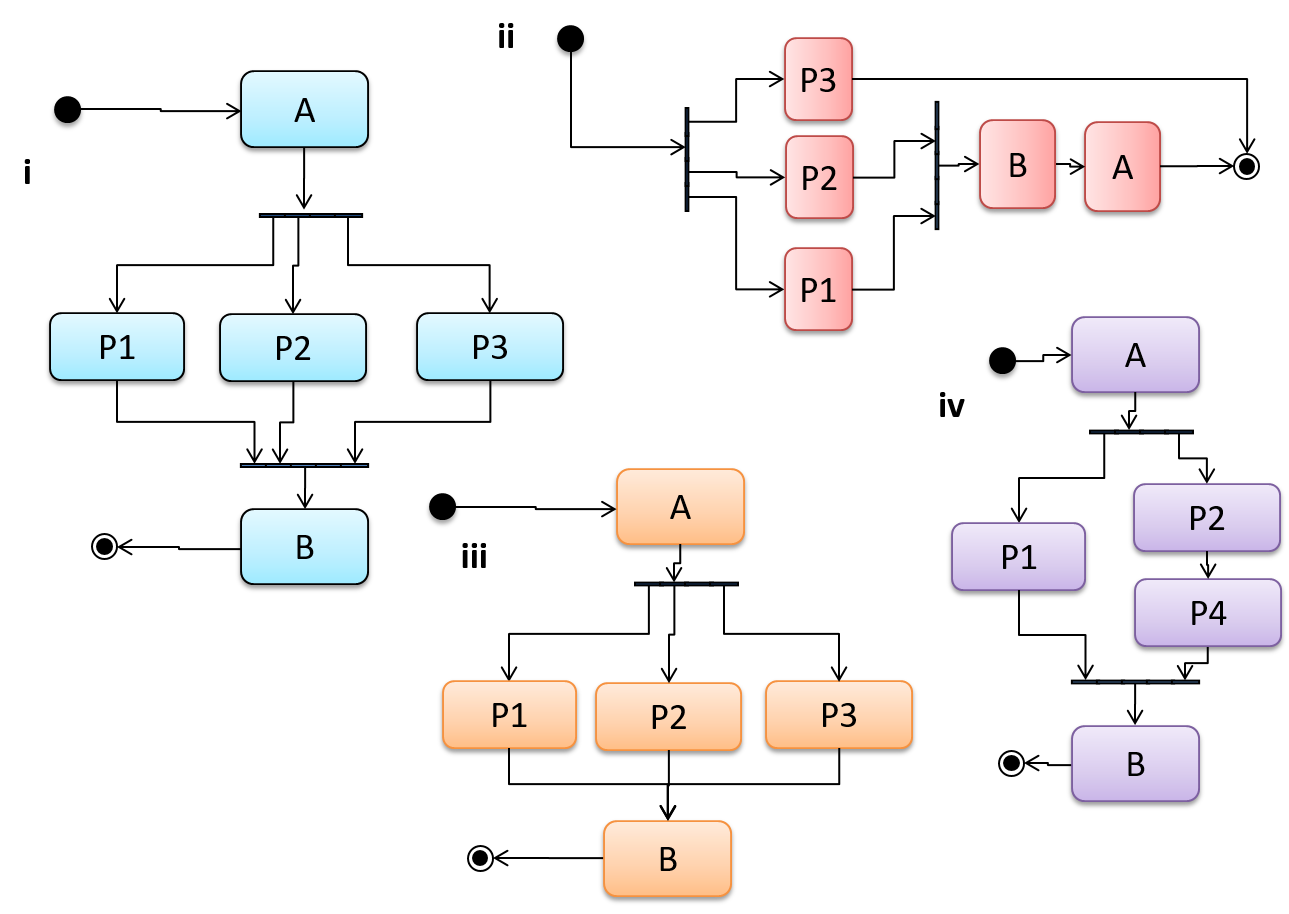• i : 👍 Correct. There can be more than two parallel paths.
• ii : Incorrect. All parallel paths that started from a fork should end in the same join node.
• iii : Incorrect. Parallel paths must end with a join node.
• iv : 👍 Correct. A parallel path can have multiple actions.

#### Rakes

Can use rakes in activity diagrams

The rake notation is used to indicate that a part of the activity is given as a separate diagram.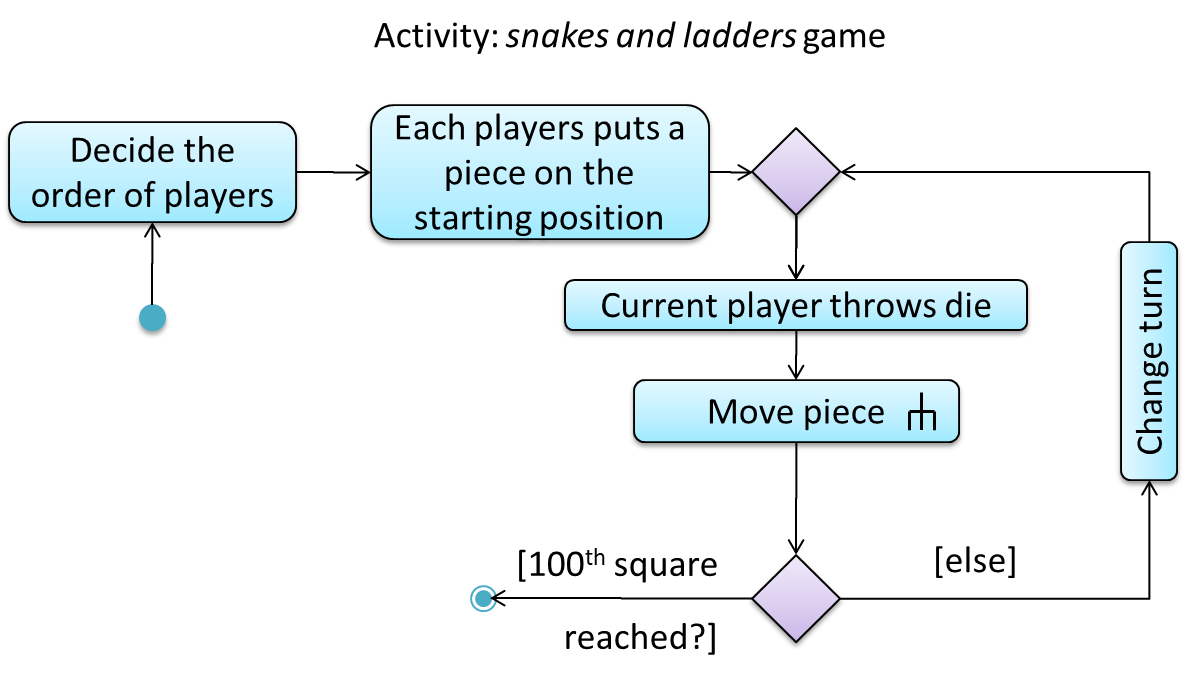The rake symbol (in the `Move piece` action above) is used to show that the action is described in another subsidiary activity diagram elsewhere. That diagram is given below.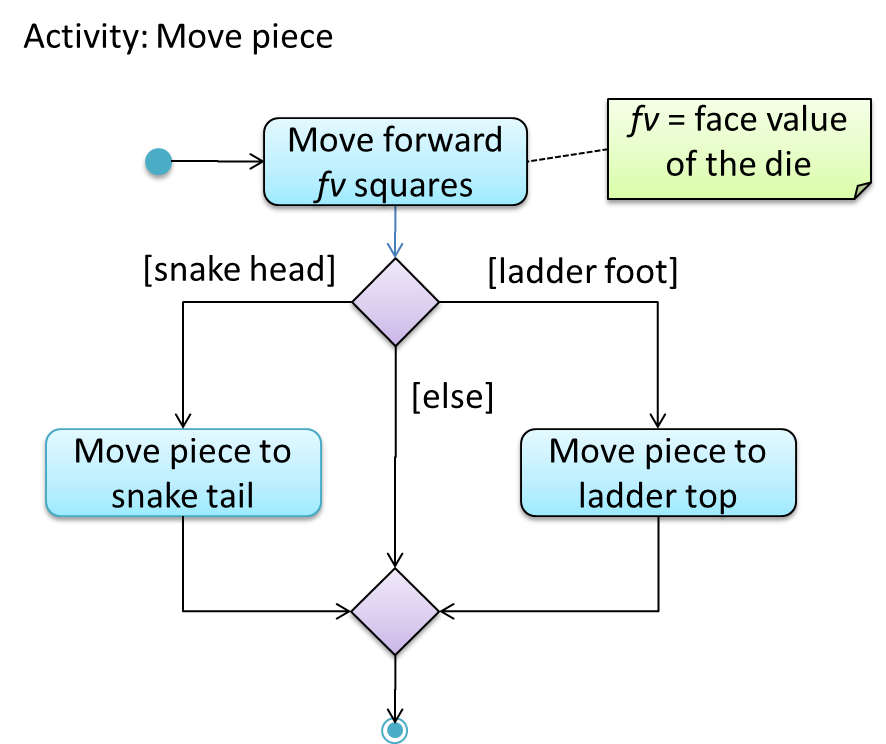#### Swimlanes

Can explain swimlanes in activity diagrams

It is possible to partition an activity diagram to show who is doing which action. Such partitioned activity diagrams are sometime called swimlane diagrams.

A simple example of a swimlane diagram: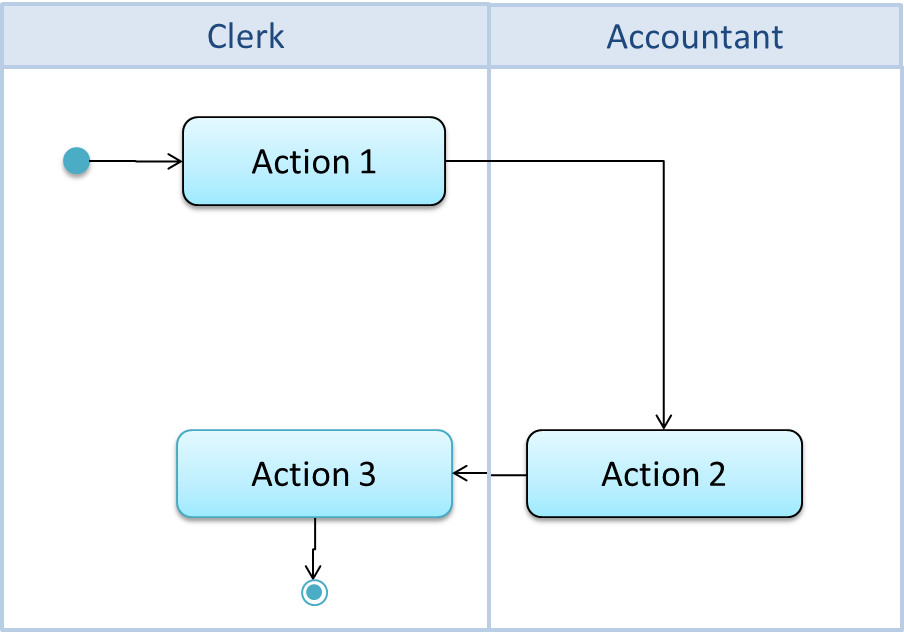#### Notes

Can use UML notes

UML notes can augment UML diagrams with additional information. These notes can be shown connected to a particular element in the diagram or can be shown without a connection. The diagram below shows examples of both.

Example: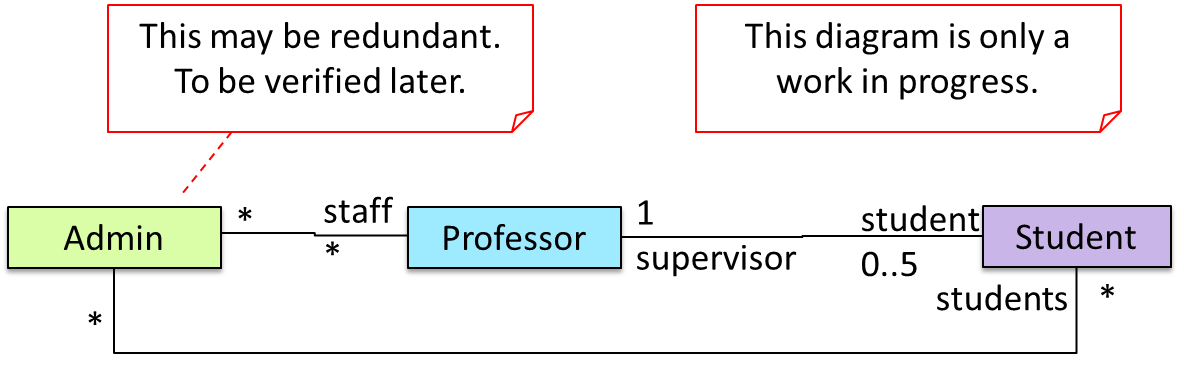#### Constraints

Can specify constraints in UML diagrams

A constraint can be given inside a note, within curly braces. Natural language or a formal notation such as OCL (Object Constraint Language) may be used to specify constraints.

Example: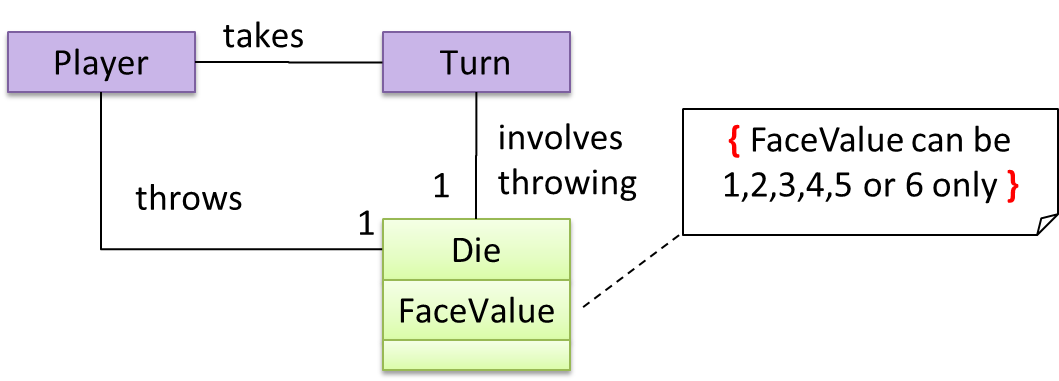## Miscellaneous

#### Object vs Class Diagrams

Can distinguish between class diagrams and object diagrams

Compared to the notation for a class diagrams, object diagrams differ in the following ways:

• Shows objects instead of classes:
• Instance name may be shown
• There is a `:` before the class name
• Instance and class names are underlined
• Methods are omitted
• Multiplicities are omitted

Furthermore, multiple object diagrams can correspond to a single class diagram.

Both object diagrams are derived from the same class diagram shown earlier. In other words, each of these object diagrams shows ‘an instance of’ the same class diagram.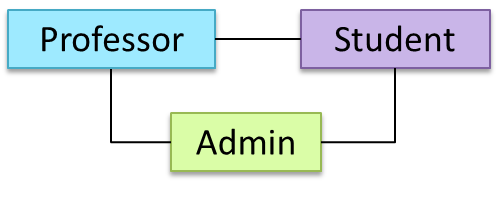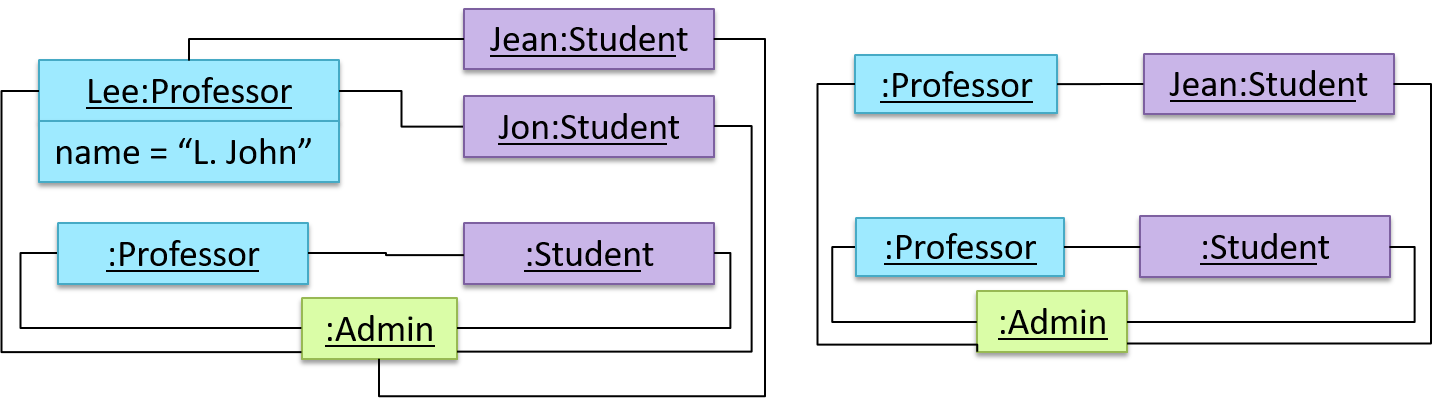Which of these class diagrams match the given object diagram?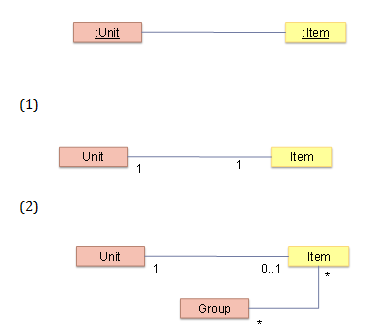• a
• b

(a) (b)

Explanation: Both class diagrams allow one `Unit` object to be linked to one `Item` object.